Browse by Topic
Related Topics

# Announcing the Rule 30 Prizes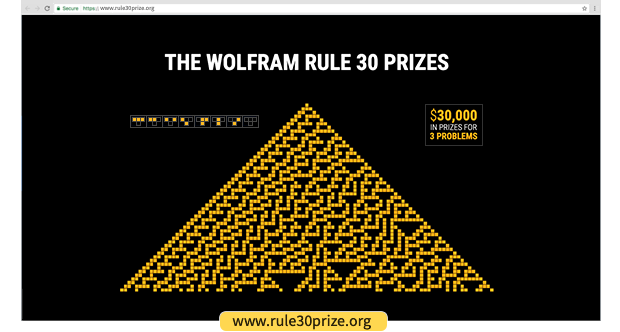## The Story of Rule 30

How can something that simple produce something that complex? It’s been nearly 40 years since I first saw rule 30—but it still amazes me. Long ago it became my personal all-time favorite science discovery, and over the years it’s changed my whole worldview and led me to all sorts of science, technology, philosophy and more.

But even after all these years, there are still many basic things we don’t know about rule 30. And I’ve decided that it’s now time to do what I can to stimulate the process of finding more of them out. So as of today, I am offering \$30,000 in prizes for the answers to three basic questions about rule 30.

The setup for rule 30 is extremely simple. One’s dealing with a sequence of lines of black and white cells. And given a particular line of black and white cells, the colors of the cells on the line below are determined by looking at each cell and its immediate neighbors and then applying the following simple rule:✕ `RulePlot[CellularAutomaton]`

If you start with a single black cell, what will happen? One might assume—as I at first did—that the rule is simple enough that the pattern it produces must somehow be correspondingly simple. But if you actually do the experiment, here’s what you find happens over the first 50 steps: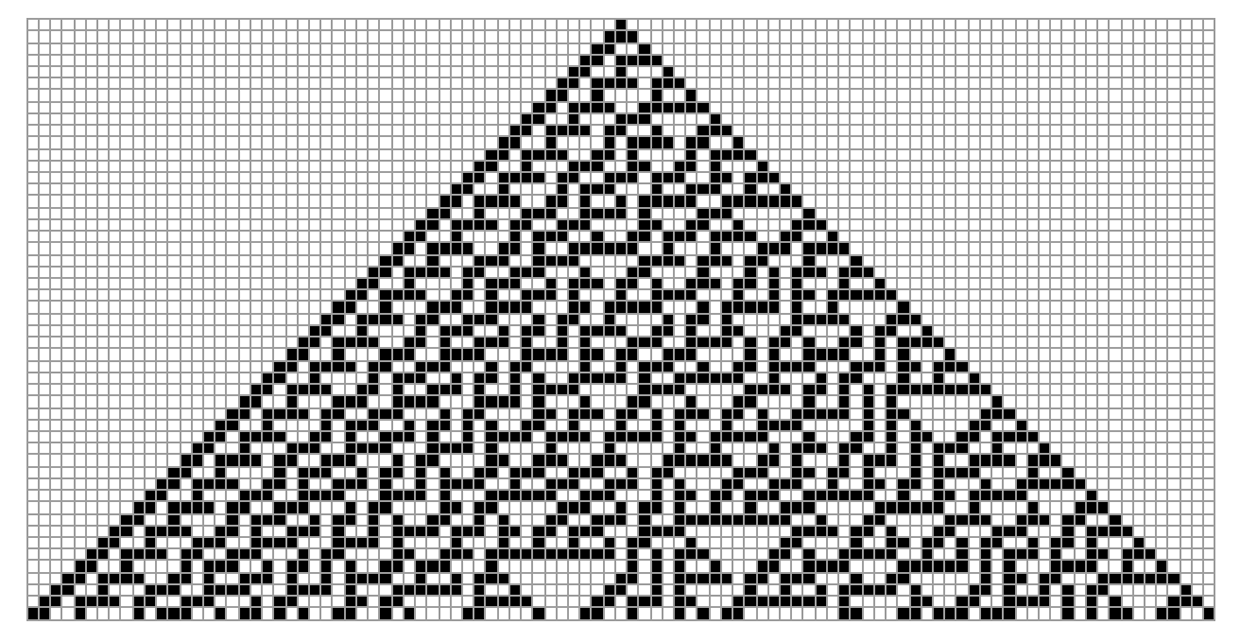✕ ```RulePlot[CellularAutomaton, {{1}, 0}, 50, Mesh -> All, ImageSize -> Full]```

But surely, one might think, this must eventually resolve into something much simpler. Yet here’s what happens over the first 300 steps:

And, yes, there’s some regularity over on the left. But many aspects of this pattern look for all practical purposes random. It’s amazing that a rule so simple can produce behavior that’s so complex. But I’ve discovered that in the computational universe of possible programs this kind of thing is common, even ubiquitous. And I’ve built a whole new kind of science—with all sorts of principles—based on this.

And gradually there’s been more and more evidence for these principles. But what specifically can rule 30 tell us? What concretely can we say about how it behaves? Even the most obvious questions turn out to be difficult. And after decades without answers, I’ve decided it’s time to define some specific questions about rule 30, and offer substantial prizes for their solutions.

I did something similar in 2007, putting a prize on a core question about a particular Turing machine. And at least in that case the outcome was excellent. In just a few months, the prize was won—establishing forever what the simplest possible universal Turing machine is, as well as providing strong further evidence for my general Principle of Computational Equivalence.

The Rule 30 Prize Problems again get at a core issue: just how complex really is the behavior of rule 30? Each of the problems asks this in a different, concrete way. Like rule 30 itself, they’re all deceptively simple to state. Yet to solve any of them will be a major achievement—that will help illuminate fundamental principles about the computational universe that go far beyond the specifics of rule 30.

I’ve wondered about every one of the problems for more than 35 years. And all that time I’ve been waiting for the right idea, or the right kind of mathematical or computational thinking, to finally be able to crack even one of them. But now I want to open this process up to the world. And I’m keen to see just what can be achieved, and what methods it will take.

## The Rule 30 Prize Problems

For the Rule 30 Prize Problems, I’m concentrating on a particularly dramatic feature of rule 30: the apparent randomness of its center column of cells. Start from a single black cell, then just look down the sequence of values of this cell—and it seems random: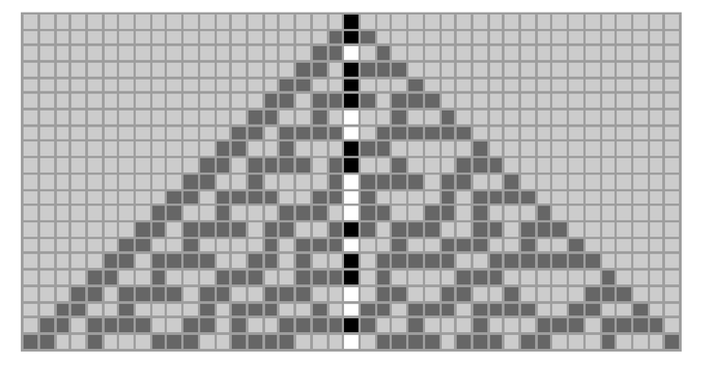✕ ```ArrayPlot[ MapIndexed[If[#2[] != 21, # /. {0 -> 0.2, 1 -> .6}, #] &, CellularAutomaton[30, {{1}, 0}, 20], {2}], Mesh -> All]```

But in what sense is it really random? And can one prove it? Each of the Prize Problems in effect uses a different criterion for randomness, then asks whether the sequence is random according to that criterion.

### Problem 1: Does the center column always remain non-periodic?

Here’s the beginning of the center column of rule 30: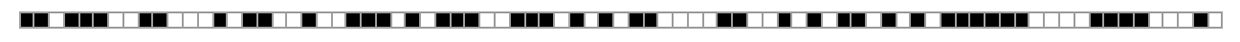✕ ```ArrayPlot[List@CellularAutomaton[30, {{1}, 0}, {80, {{0}}}], Mesh -> True, ImageSize -> Full]```

It’s easy to see that this doesn’t repeat—it doesn’t become periodic. But this problem is about whether the center column ever becomes periodic, even after an arbitrarily large number of steps. Just by running rule 30, we know the sequence doesn’t become periodic in the first billion steps. But what about ever? To establish that, we need a proof. (Here are the first million and first billion bits in the sequence, by the way, as entries in the Wolfram Data Repository.)

### Problem 2: Does each color of cell occur on average equally often in the center column?

Here’s what one gets if one tallies the number of black and of white cells in successively more steps in the center column of rule 30: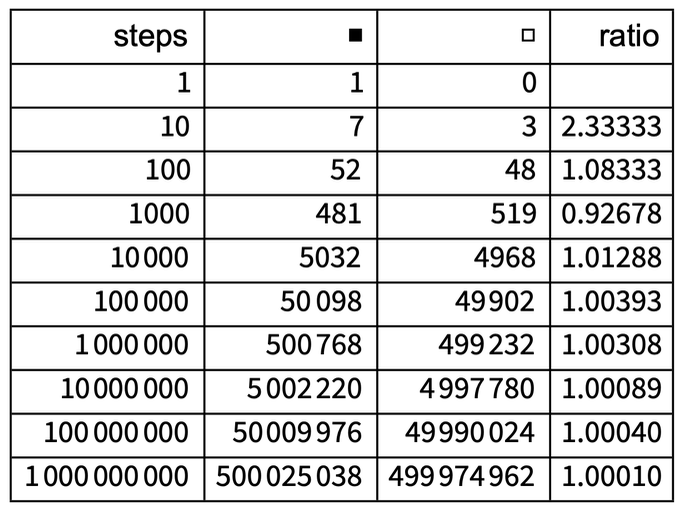✕ ```Dataset[{{1, 1, 0, ""}, {10, 7, 3, 2.3333333333333335}, {100, 52, 48, 1.0833333333333333}, {1000, 481, 519, 0.9267822736030829}, {10000, 5032, 4968, 1.0128824476650564}, {100000, 50098, 49902, 1.0039276982886458}, {1000000, 500768, 499232, 1.003076725850907}, {10000000, 5002220, 4997780, 1.0008883944471345}, {100000000, 50009976, 49990024, 1.000399119632349}, {1000000000, 500025038, 499974962, 1.0001001570154626}}]```

The results are certainly close to equal for black vs. white. But what this problem asks is whether the limit of the ratio after an arbitrarily large number of steps is exactly 1.

### Problem 3: Does computing the nth cell of the center column require at least O(n) computational effort?

To find the nth cell in the center column, one can always just run rule 30 for n steps, computing the values of all the cells in this diamond: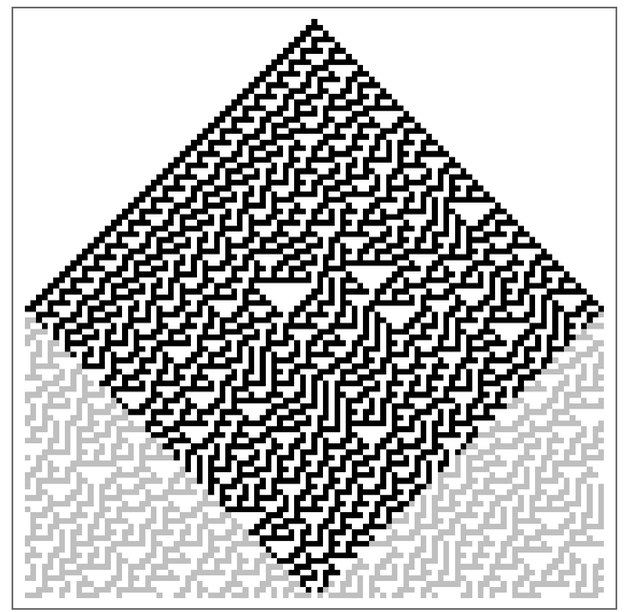✕ ```With[{n = 100}, ArrayPlot[ MapIndexed[If[Total[Abs[#2 - n/2 - 1]] <= n/2, #, #/4] &, CellularAutomaton[30, CenterArray[{1}, n + 1], n], {2}]]]```

But if one does this directly, one’s doing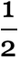n2 individual cell updates, so the computational effort required goes up like O(n2). This problem asks if there’s a shortcut way to compute the value of the nth cell, without all this intermediate computation—or, in particular, in less than O(n) computational effort.

## The Digits of Pi

Rule 30 is a creature of the computational universe: a system found by exploring possible simple programs with the new intellectual framework that the paradigm of computation provides. But the problems I’ve defined about rule 30 have analogs in mathematics that are centuries old.

Consider the digits of π. They’re a little like the center column of rule 30. There’s a definite algorithm for generating them. Yet once generated they seem for all practical purposes random: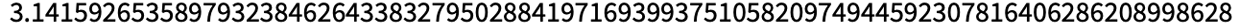✕ `N[Pi, 85]`

Just to make the analog a little closer, here are the first few digits of π in base 2: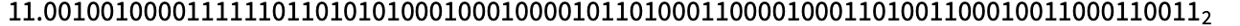✕ `BaseForm[N[Pi, 25], 2]`

And here are the first few bits in the center column of rule 30: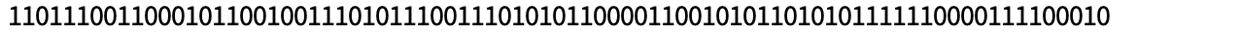✕ `Row[CellularAutomaton[30, {{1}, 0}, {90, {{0}}}]]`

Just for fun, one can convert these to base 10: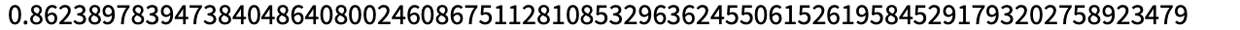✕ ```N[FromDigits[{Flatten[CellularAutomaton[30, {{1}, 0}, {500, {0}}]], 0}, 2], 85]```

Of course, the known algorithms for generating the digits of π are considerably more complicated than the simple rule for generating the center column of rule 30. But, OK, so what’s known about the digits of π?

Well, we know they don’t repeat. That was proved in the 1760s when it was shown that π is an irrational number—because the only numbers whose digits repeat are rational numbers. (It was also shown in 1882 that π is transcendental, i.e. that it cannot be expressed in terms of roots of polynomials.)

How about the analog of problem 2? Do we know if in the digit sequence of π different digits occur with equal frequency? By now more than 100 trillion binary digits have been computed—and the measured frequencies of digits are very close (in the first 40 trillion binary digits the ratio of 1s to 0s is about 0.9999998064). But in the limit, are the frequencies exactly the same? People have been wondering about this for several centuries. But so far mathematics hasn’t succeeded in delivering any results.

For rational numbers, digit sequences are periodic, and it’s easy to work out relative frequencies of digits. But for the digit sequences of all other “naturally constructed” numbers, basically there’s nothing known about limiting frequencies of digits. It’s a reasonable guess that actually the digits of π (as well as the center column of rule 30) are “normal”, in the sense that not only every individual digit, but also every block of digits of any given length in the limit occur with equal frequency. And as was noted in the 1930s, it’s perfectly possible to “digit-construct” normal numbers. Champernowne’s number, formed by concatenating the digits of successive integers, is an example (and, yes, this works in any base, and one can also get normal numbers by concatenating values of functions of successive integers):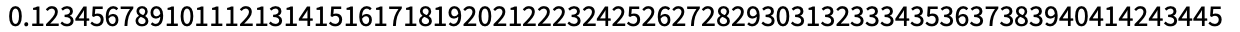✕ `N[ChampernowneNumber, 85]`

But the point is that for “naturally constructed” numbers formed by combinations of standard mathematical functions, there’s simply no example known where any regularity of digits has been found. Of course, it ultimately depends what one means by “regularity”—and at some level the problem devolves into a kind of number-digit analog of the search for extraterrestrial intelligence. But there’s absolutely no proof that one couldn’t, for example, find even some strange combination of square roots that would have a digit sequence with some very obvious regularity.

OK, so what about the analog of problem 3 for the digits of π? Unlike rule 30, where the obvious way to compute elements in the sequence is one step at a time, traditional ways of computing digits of π involve getting better approximations to π as a complete number. With the standard (bizarre-looking) series invented by Ramanujan in 1910 and improved by the Chudnovsky brothers in 1989, the first few terms in the series give the following approximations: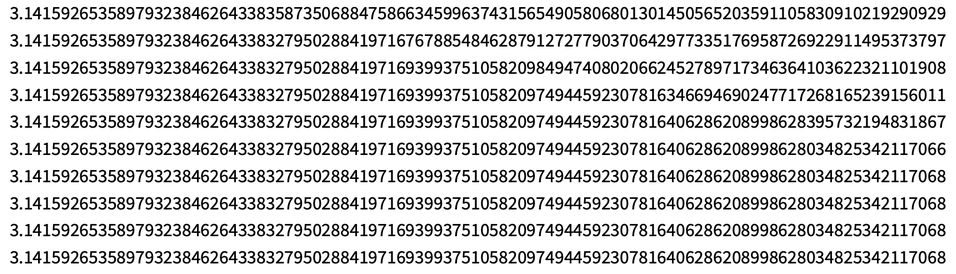✕ ```Style[Table[N[(12*\!\( \*UnderoverscriptBox[\(\[Sum]\), \(k = 0\), \(n\)] \*FractionBox[\( \*SuperscriptBox[\((\(-1\))\), \(k\)]*\(\((6*k)\)!\)*\((13591409 + 545140134*k)\)\), \(\(\((3*k)\)!\) \*SuperscriptBox[\((\(k!\))\), \(3\)]* \*SuperscriptBox[\(640320\), \(3*k + 3/2\)]\)]\))^-1, 100], {n, 10}] // Column, 9]```

So how much computational effort is it to find the nth digit? The number of terms required in the series is O(n). But each term needs to be computed to n-digit precision, which requires at least O(n) individual digit operations—implying that altogether the computational effort required is more than O(n).

Until the 1990s it was assumed that there wasn’t any way to compute the nth digit of π without computing all previous ones. But in 1995 Simon Plouffe discovered that actually it’s possible to compute—albeit slightly probabilistically—the nth digit without computing earlier ones. And while one might have thought that this would allow the nth digit to be obtained with less than O(n) computational effort, the fact that one has to do computations at n-digit precision means that at least O(n) computational effort is still required.

## Results, Analogies and Intuitions

### Problem 1: Does the center column always remain non-periodic?

Of the three Rule 30 Prize Problems, this is the one on which the most progress has already been made. Because while it’s not known if the center column in the rule 30 pattern ever becomes periodic, Erica Jen showed in 1986 that no two columns can both become periodic. And in fact, one can also give arguments that a single column plus scattered cells in another column can’t both be periodic.

The proof about a pair of columns uses a special feature of rule 30. Consider the structure of the rule: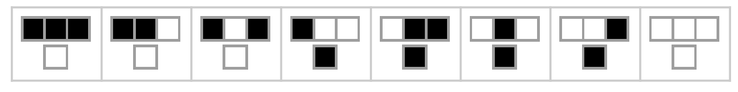✕ `RulePlot[CellularAutomaton]`

Normally one would just say that given each triple of cells, the rule determines the color of the center cell below. But for rule 30, one can effectively also run the rule sideways: given the cell to the right and above, one can also uniquely determine the color of the cell to the left. And what this means is that if one is given two adjacent columns, it’s possible to reconstruct the whole pattern to the left: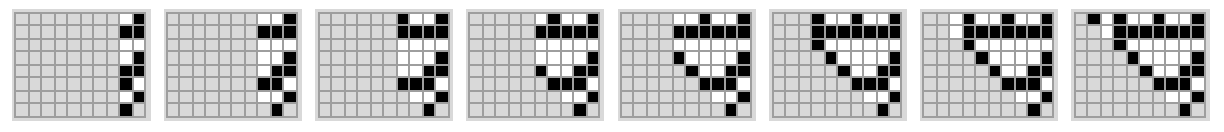✕ ```GraphicsRow[ ArrayPlot[#, PlotRange -> 1, Mesh -> All, PlotRange -> 1, Background -> LightGray, ImageSize -> {Automatic, 80}] & /@ (PadLeft[#, {Length[#], 10}, 10] & /@ Module[{data = {{0, 1}, {1, 1}, {0, 0}, {0, 1}, {1, 1}, {1, 0}, {0, 1}, {1, 10}}}, Flatten[{{data}, Table[Join[ Table[Module[{p, q = data[[n, 1]], r = data[[n, 2]], s = data[[n + 1, 1]] }, p = Mod[-q - r - q r + s, 2]; PrependTo[data[[n]], p]], {n, 1, Length[data] - i}], PrependTo[data[[-#]], 10] & /@ Reverse[Range[i]]], {i, 7}]}, 1]])]```

But if the columns were periodic, it immediately follows that the reconstructed pattern would also have to be periodic. Yet by construction at least the initial condition is definitely not periodic, and hence the columns cannot both be periodic. The same argument works if the columns are not adjacent, and if one doesn’t know every cell in both columns. But there’s no known way to extend the argument to a single column—such as the center column—and thus it doesn’t resolve the first Rule 30 Prize Problem.

OK, so what would be involved in resolving it? Well, if it turns out that the center column is eventually periodic, one could just compute it, and show that. We know it’s not periodic for the first billion steps, but one could at least imagine that there could be a trillion-step transient, after which it’s periodic.

Is that plausible? Well, transients do happen—and theoretically (just like in the classic Turing machine halting problem) they can even be arbitrarily long. Here’s a somewhat funky example—found by a search—of a rule with 4 possible colors (totalistic code 150898). Run it for 200 steps, and the center column looks quite random: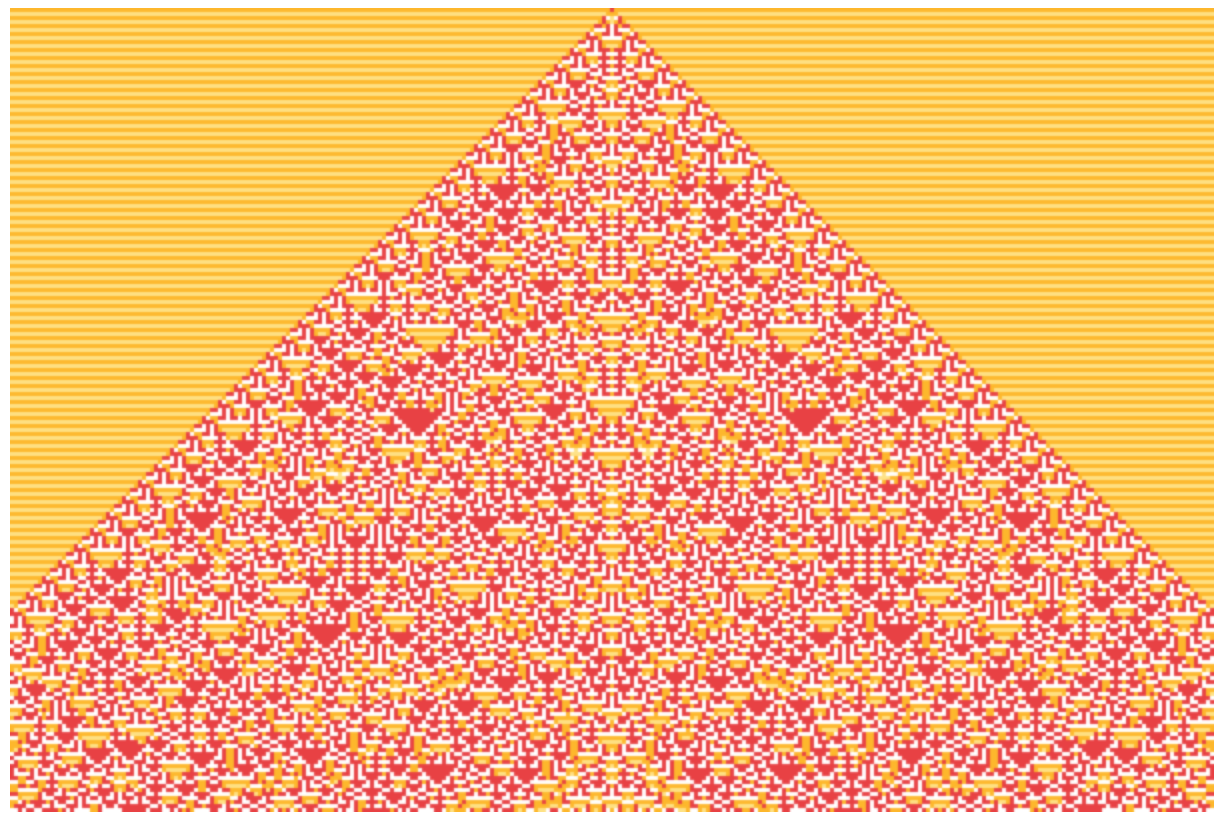✕ ```ArrayPlot[ CellularAutomaton[{150898, {4, 1}, 1}, {{1}, 0}, {200, 150 {-1, 1}}], ColorRules -> {0 -> Hue[0.12, 1, 1], 1 -> Hue[0, 0.73, 0.92], 2 -> Hue[0.13, 0.5, 1], 3 -> Hue[0.17, 0, 1]}, PixelConstrained -> 2, Frame -> False]```

After 500 steps, the whole pattern still looks quite random: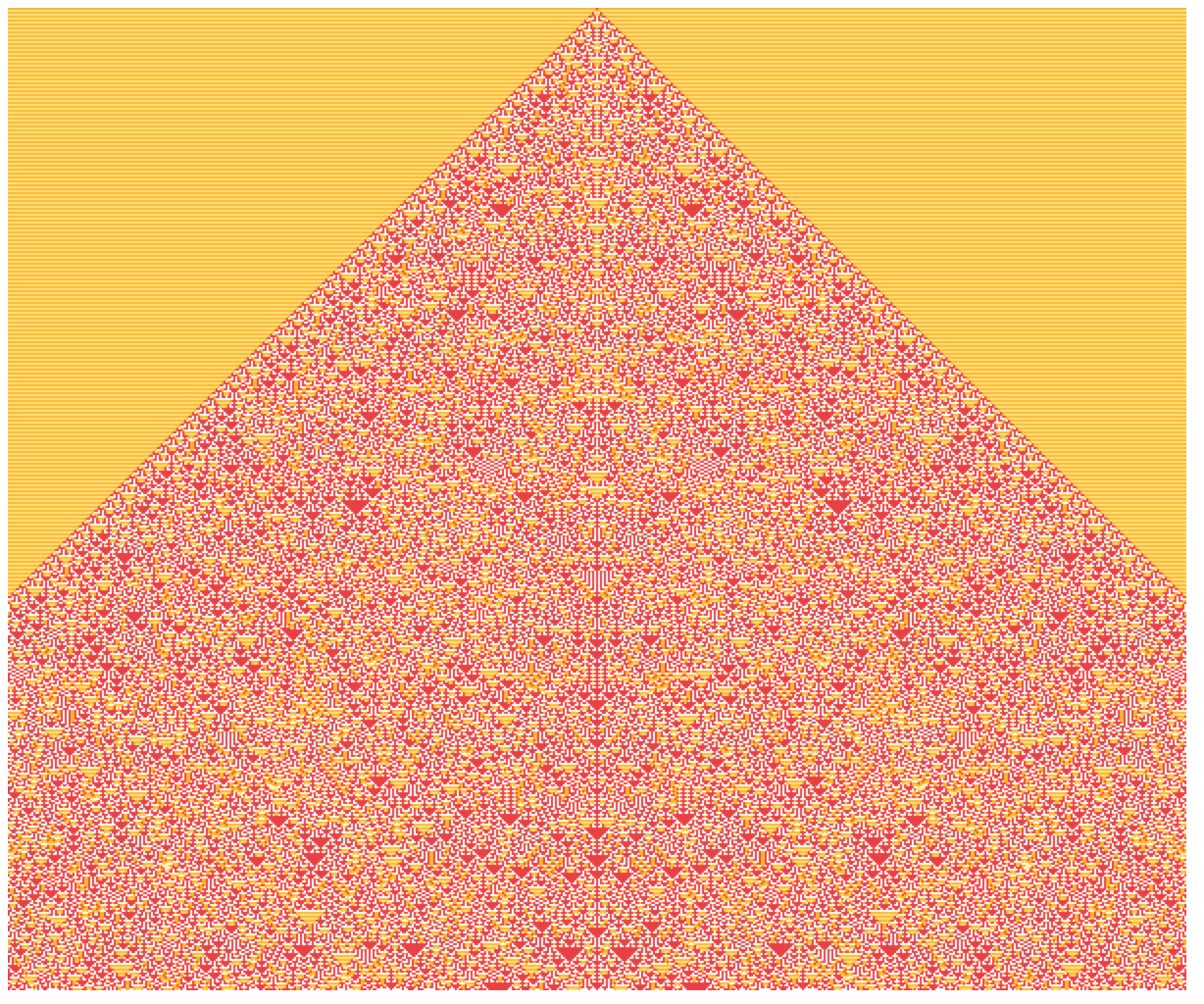✕ ```ArrayPlot[ CellularAutomaton[{150898, {4, 1}, 1}, {{1}, 0}, {500, 300 {-1, 1}}], ColorRules -> {0 -> Hue[0.12, 1, 1], 1 -> Hue[0, 0.73, 0.92], 2 -> Hue[0.13, 0.5, 1], 3 -> Hue[0.17, 0, 1]}, Frame -> False, ImagePadding -> 0, PlotRangePadding -> 0, PixelConstrained -> 1]```

But if one zooms in around the center column, there’s something surprising: after 251 steps, the center column seems to evolve to a fixed value (or at least it’s fixed for more than a million steps):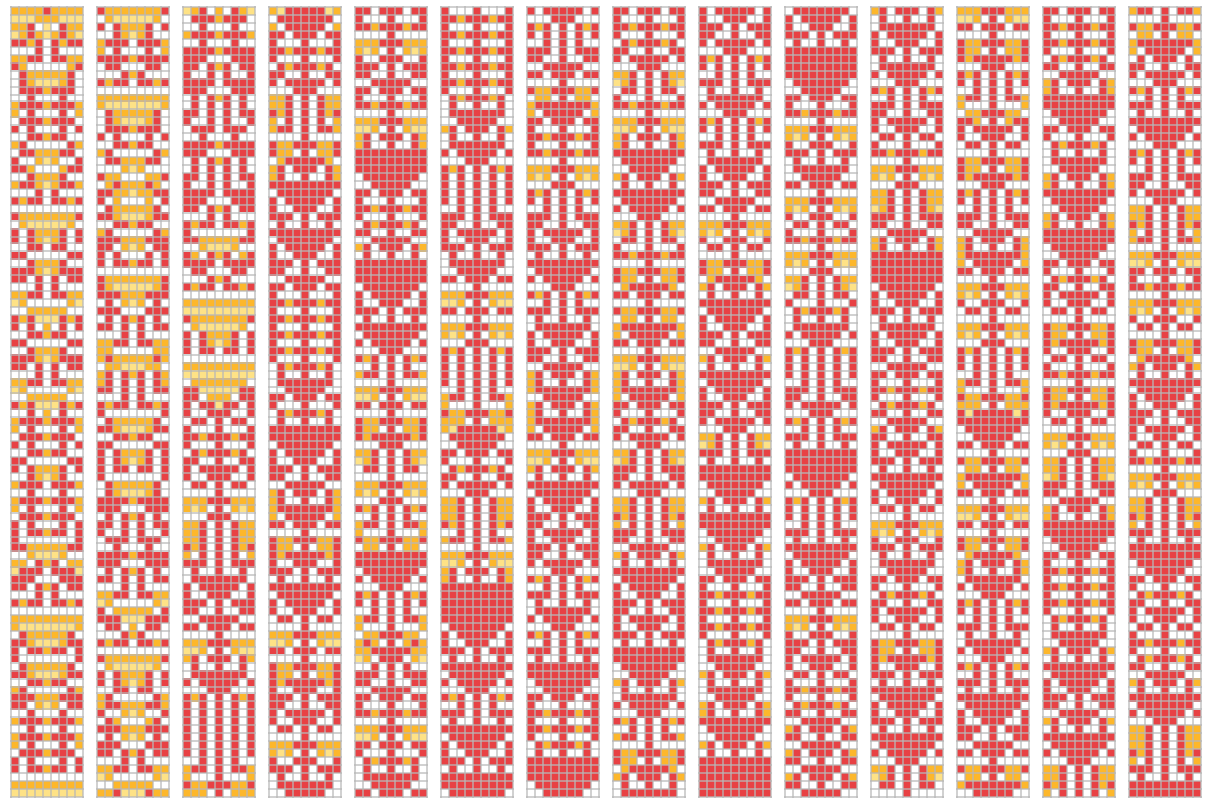✕ ```Grid[{ArrayPlot[#, Mesh -> True, ColorRules -> {0 -> Hue[0.12, 1, 1], 1 -> Hue[0, 0.73, 0.92], 2 -> Hue[0.13, 0.5, 1], 3 -> Hue[0.17, 0, 1]}, ImageSize -> 38, MeshStyle -> Lighter[GrayLevel[.5, .65], .45]] & /@ Partition[ CellularAutomaton[{150898, {4, 1}, 1}, {{1}, 0}, {1400, {-4, 4}}], 100]}, Spacings -> .35]```

Could some transient like this happen in rule 30? Well, take a look at the rule 30 pattern, now highlighting where the diagonals on the left are periodic: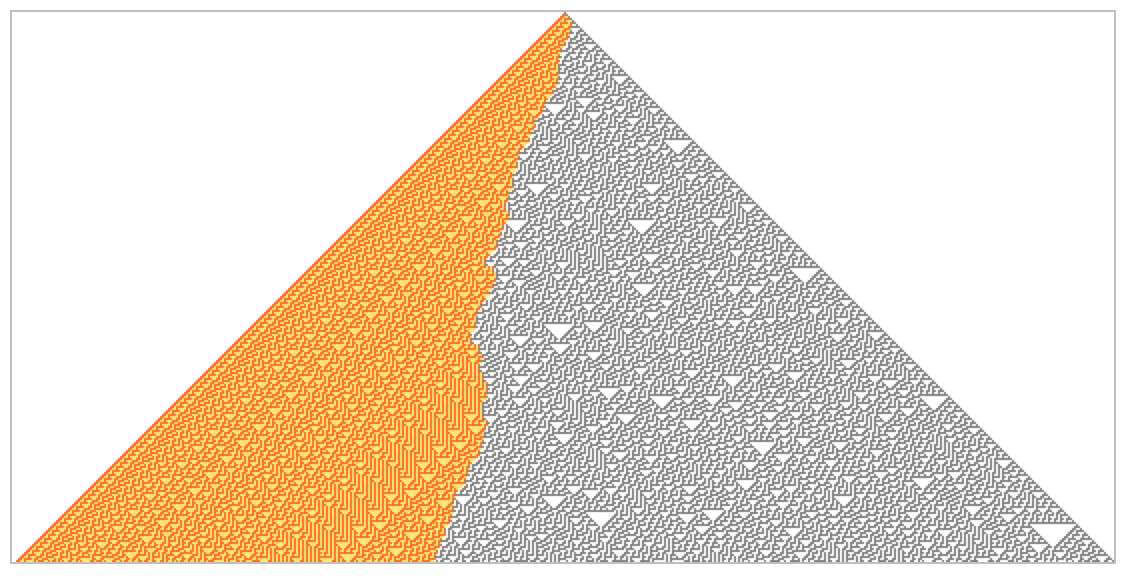✕ ```steps = 500; diagonalsofrule30 = Reverse /@ Transpose[ MapIndexed[RotateLeft[#1, (steps + 1) - #2[]] &, CellularAutomaton[30, {{1}, 0}, steps]]]; diagonaldataofrule30 = Table[With[{split = Split[Partition[Drop[diagonalsofrule30[[k]], 1], 8]], ones = Flatten[ Position[Reverse[Drop[diagonalsofrule30[[k]], 1]], 1]]}, {Length[split[]], split[[1, 1]], If[Length[split] > 1, split[[2, 1]], Length[diagonalsofrule30[[k]]] - Floor[k/2]]}], {k, 1, 2 steps + 1}]; transientdiagonalrule30 = %; transitionpointofrule30 = If[IntegerQ[#[]], #[], If[#[] > 1, 8 #[] + Count[Split[#[] - #[]][], 0] + 1, 0] ] & /@ diagonaldataofrule30; decreasingtransitionpointofrule30 = Append[Min /@ Partition[transitionpointofrule30, 2, 1], 0]; transitioneddiagonalsofrule30 = Table[Join[ Take[diagonalsofrule30[[n]], decreasingtransitionpointofrule30[[n]]] + 2, Drop[diagonalsofrule30[[n]], decreasingtransitionpointofrule30[[n]]]], {n, 1, 2 steps + 1}]; transientdiagonalrule30 = MapIndexed[RotateRight[#1, (steps + 1) - #2[]] &, Transpose[Reverse /@ transitioneddiagonalsofrule30]]; smallertransientdiagonalrule30 = Take[#, {225, 775}] & /@ Take[transientdiagonalrule30, 275]; Framed[ArrayPlot[smallertransientdiagonalrule30, ColorRules -> {0 -> White, 1 -> Gray, 2 -> Hue[0.14, 0.55, 1], 3 -> Hue[0.07, 1, 1]}, PixelConstrained -> 1, Frame -> None, ImagePadding -> 0, ImageMargins -> 0, PlotRangePadding -> 0, PlotRangePadding -> Full ], FrameMargins -> 0, FrameStyle -> GrayLevel[.75]]```

There seems to be a boundary that separates order on the left from disorder on the right. And at least over the first 100,000 or so steps, the boundary seems to move on average about 0.252 steps to the left at each step—with roughly random fluctuations: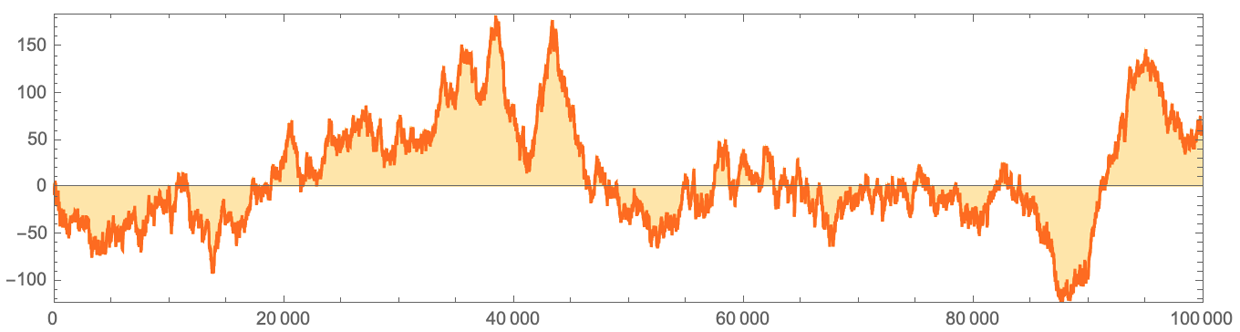✕ ```data = CloudGet[ CloudObject[ "https://www.wolframcloud.com/obj/bc470188-f629-4497-965d-\ a10fe057e2fd"]]; ListLinePlot[ MapIndexed[{First[#2], -# - .252 First[#2]} &, Module[{m = -1, w}, w = If[First[#] > m, m = First[#], m] & /@ data[]; m = 1; Table[While[w[[m]] < i, m++]; m - i, {i, 100000}]]], Filling -> Axis, AspectRatio -> 1/4, MaxPlotPoints -> 10000, Frame -> True, PlotRangePadding -> 0, AxesOrigin -> {Automatic, 0}, PlotStyle -> Hue[0.07`, 1, 1], FillingStyle -> Directive[Opacity[0.35`], Hue[0.12`, 1, 1]]]```

But how do we know that there won’t at some point be a huge fluctuation, that makes the order on the left cross the center column, and perhaps even make the whole pattern periodic? From the data we have so far, it looks unlikely, but I don’t know any way to know for sure.

And it’s certainly the case that there are systems with exceptionally long “transients”. Consider the distribution of primes, and compute LogIntegral[n] - PrimePi[n]: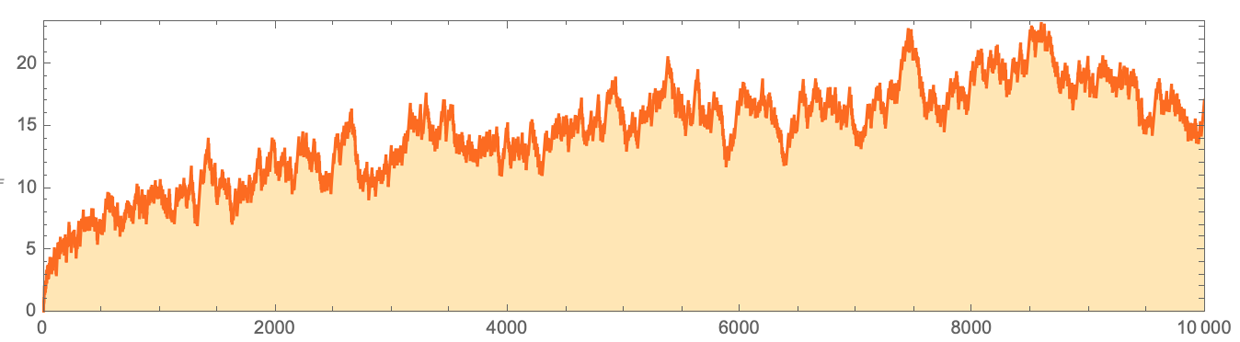✕ ```DiscretePlot[LogIntegral[n] - PrimePi[n], {n, 10000}, Filling -> Axis, Frame -> True, PlotRangePadding -> 0, AspectRatio -> 1/4, Joined -> True, PlotStyle -> Hue[0.07`, 1, 1], FillingStyle -> Directive[Opacity[0.35`], Hue[0.12`, 1, 1]]]```

Yes, there are fluctuations. But from this picture it certainly looks as if this difference is always going to be positive. And that’s, for example, what Ramanujan thought. But it turns out it isn’t true. At first the bound for where it would fail was astronomically large (Skewes’s number 10^10^10^964). And although still nobody has found an explicit value of n for which the difference is negative, it’s known that before n = 10317 there must be one (and eventually the difference will be negative at least nearly a millionth of the time).

I strongly suspect that nothing like this happens with the center column of rule 30. But until we have a proof that it can’t, who knows?

One might think, by the way, that while one might be able to prove periodicity by exposing regularity in the center column of rule 30, nothing like that would be possible for non-periodicity. But actually, there are patterns whose center columns one can readily see are non-periodic, even though they’re very regular. The main class of examples are nested patterns. Here’s a very simple example, from rule 161—in which the center column has white cells when n = 2k: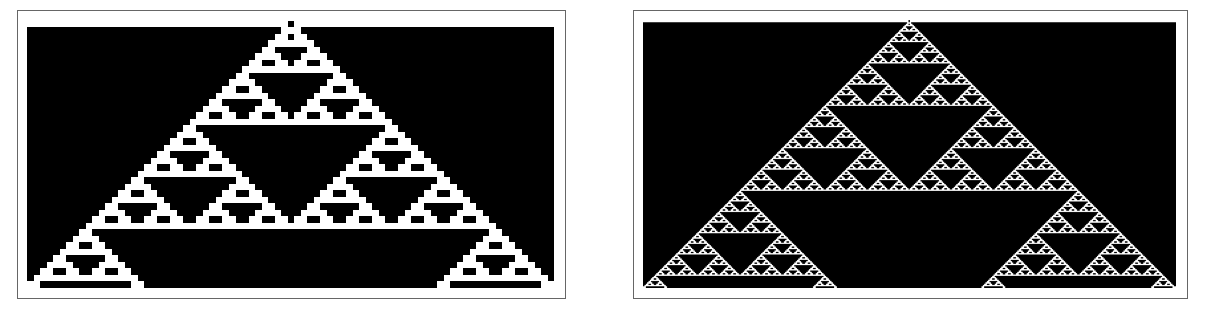✕ ```GraphicsRow[ ArrayPlot[CellularAutomaton[161, {{1}, 0}, #]] & /@ {40, 200}]```

Here’s a slightly more elaborate example (from the 2-neighbor 2-color rule 69540422), in which the center column is a Thue–Morse sequence ThueMorse[n]: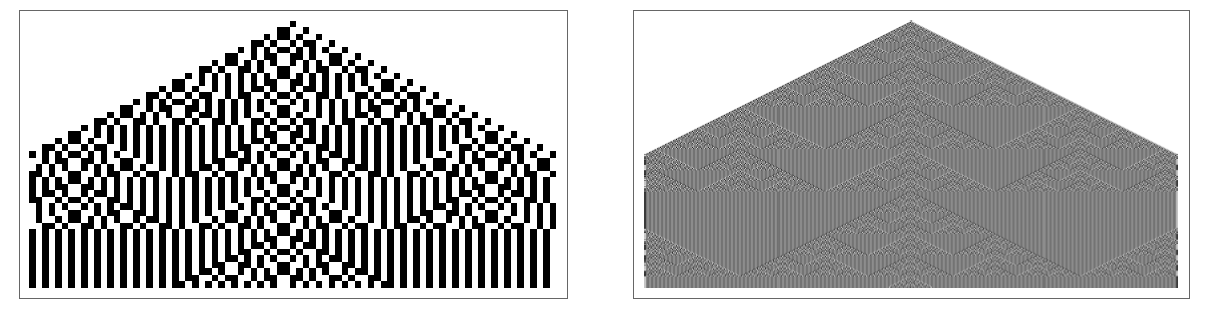✕ ```GraphicsRow[ ArrayPlot[ CellularAutomaton[{69540422, 2, 2}, {{1}, 0}, {#, {-#, #}}]] & /@ {40, 400}]```

One can think of the Thue–Morse sequence as being generated by successively applying the substitutions:✕ ```RulePlot[SubstitutionSystem[{0 -> {0, 1}, 1 -> {1, 0}}], Appearance -> "Arrow"]```

And it turns out that the nth term in this sequence is given by Mod[DigitCount[n, 2, 1], 2]—which is never periodic.

Will it turn out that the center column of rule 30 can be generated by a substitution system? Again, I’d be amazed (although there are seemingly natural examples where very complex substitution systems do appear). But once again, until one has a proof, who knows?

Here’s something else, that may be confusing, or may be helpful. The Rule 30 Prize Problems all concern rule 30 running in an infinite array of cells. But what if one considers just n cells, say with the periodic boundary conditions (i.e. taking the right neighbor of the rightmost cell to be the leftmost cell, and vice versa)? There are 2n possible total states of the system—and one can draw a state transition diagram that shows which state evolves to which other. Here’s the diagram for n = 5: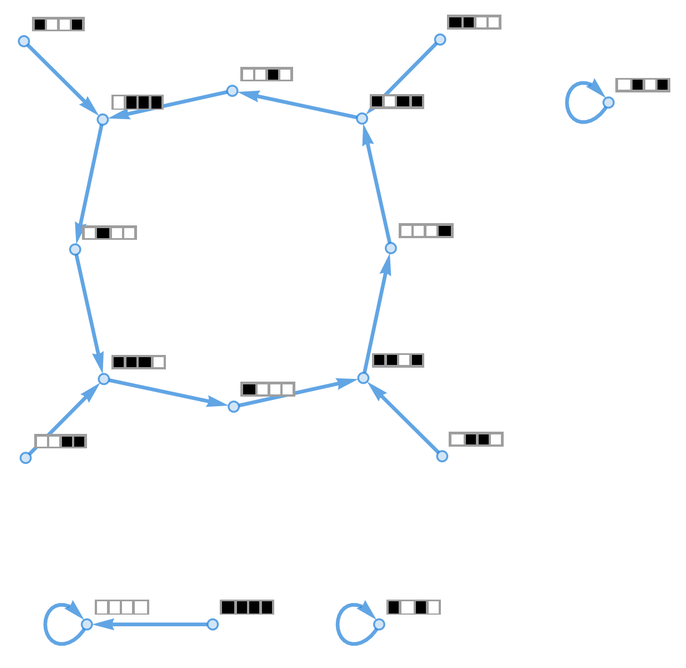✕ ```Graph[# -> CellularAutomaton[#] & /@ Tuples[{1, 0}, 4], VertexLabels -> ((# -> ArrayPlot[{#}, ImageSize -> 30, Mesh -> True]) & /@ Tuples[{1, 0}, 4])]```

And here it is for n = 4 through n = 11: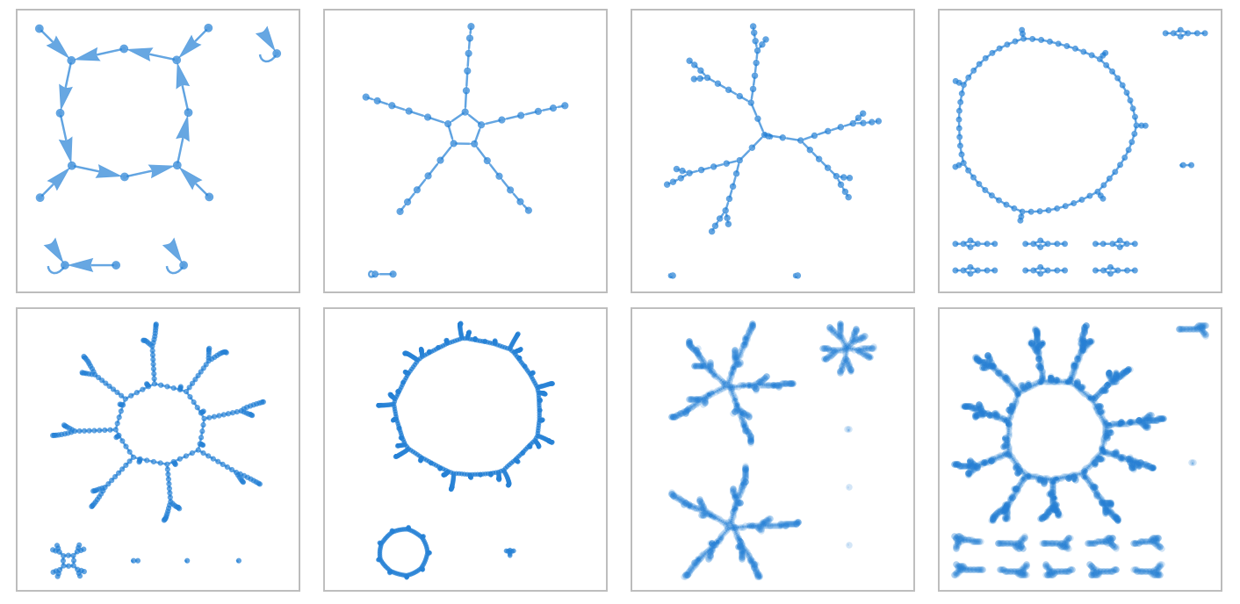✕ ```Row[Table[ Framed[Graph[# -> CellularAutomaton[#] & /@ Tuples[{1, 0}, n]]], {n, 4, 11}]]```

The structure is that there are a bunch of states that appear only as transients, together with other states that are on cycles. Inevitably, no cycle can be longer than 2n (actually, symmetry considerations show that it always has to be somewhat less than this).

OK, so on a size-n array, rule 30 always has to show behavior that becomes periodic with a period that’s less than 2n. Here are the actual periods starting from a single black cell initial condition, plotted on a log scale: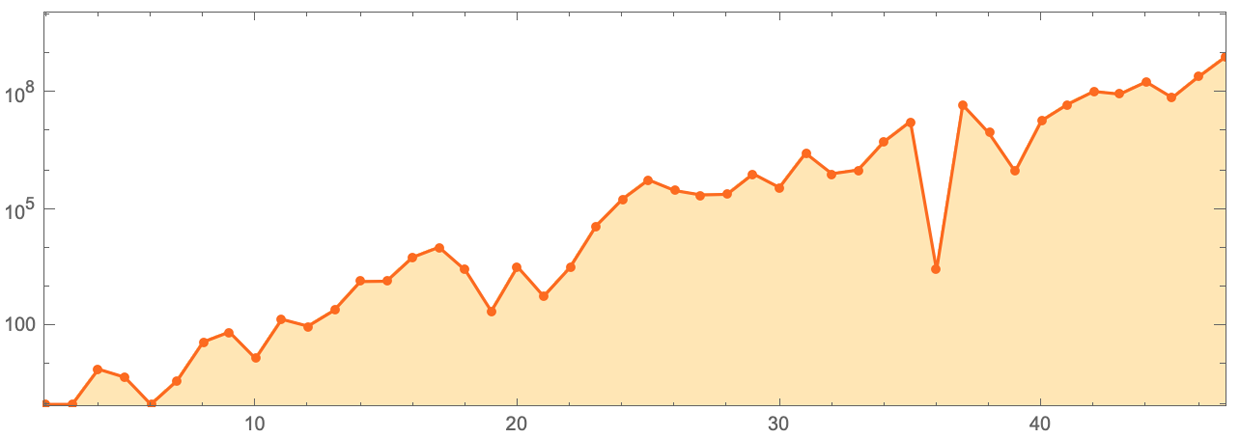✕ ```ListLogPlot[ Normal[Values[ ResourceData[ "Repetition Periods for Elementary Cellular Automata"][ Select[#Rule == 30 &]][All, "RepetitionPeriods"]]], Joined -> True, Filling -> Bottom, Mesh -> All, MeshStyle -> PointSize[.008], AspectRatio -> 1/3, Frame -> True, PlotRange -> {{47, 2}, {0, 10^10}}, PlotRangePadding -> .1, PlotStyle -> Hue[0.07`, 1, 1], FillingStyle -> Directive[Opacity[0.35`], Hue[0.12`, 1, 1]]]```

And at least for these values of n, a decent fit is that the period is about 20.63 n. And, yes, at least in all these cases, the period of the center column is equal to the period of the whole evolution. But what do these finite-size results imply about the infinite-size case? I, at least, don’t immediately see.

### Problem 2: Does each color of cell occur on average equally often in the center column?

Here’s a plot of the running excess of 1s over 0s in 10,000 steps of the center column of rule 30: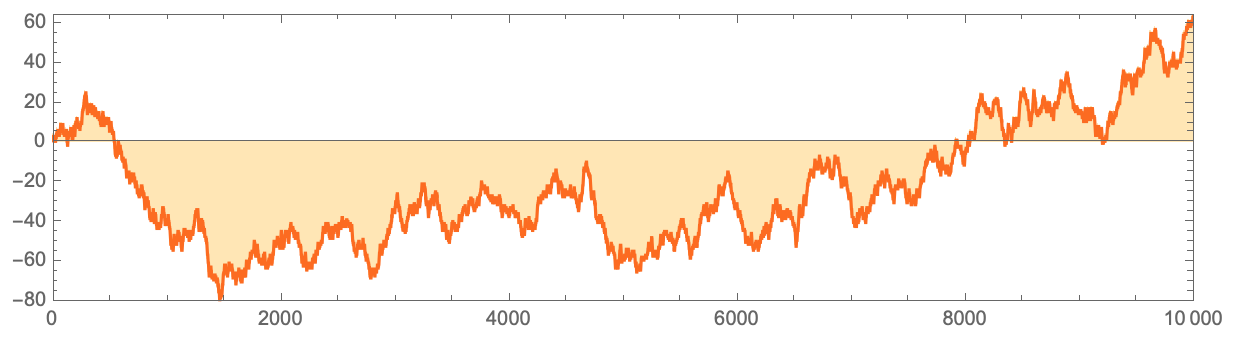✕ ```ListLinePlot[ Accumulate[2 CellularAutomaton[30, {{1}, 0}, {10^4 - 1, {{0}}}] - 1], AspectRatio -> 1/4, Frame -> True, PlotRangePadding -> 0, AxesOrigin -> {Automatic, 0}, Filling -> Axis, PlotStyle -> Hue[0.07`, 1, 1], FillingStyle -> Directive[Opacity[0.35`], Hue[0.12`, 1, 1]]]```

Here it is for a million steps: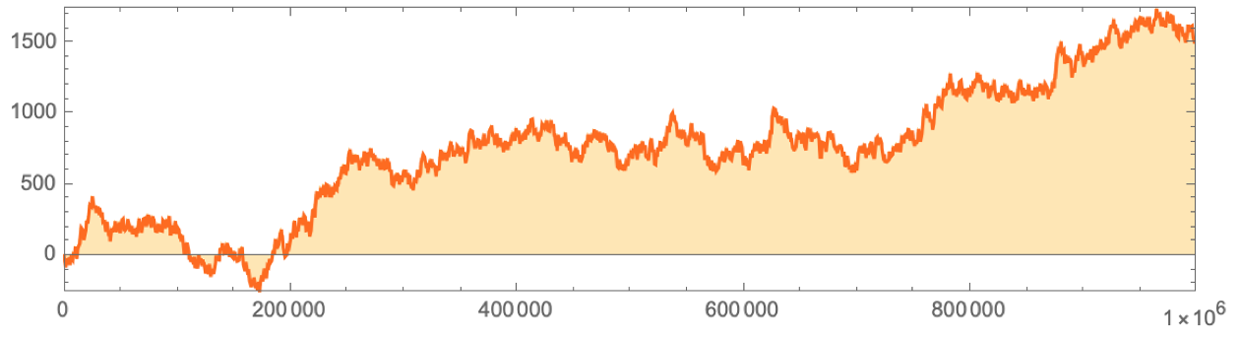✕ ```ListLinePlot[ Accumulate[ 2 ResourceData[ "A Million Bits of the Center Column of the Rule 30 Cellular Automaton"] - 1], Filling -> Axis, Frame -> True, PlotRangePadding -> 0, AspectRatio -> 1/4, MaxPlotPoints -> 1000, PlotStyle -> Hue[0.07`, 1, 1], FillingStyle -> Directive[Opacity[0.35`], Hue[0.12`, 1, 1]]]```

And a billion steps: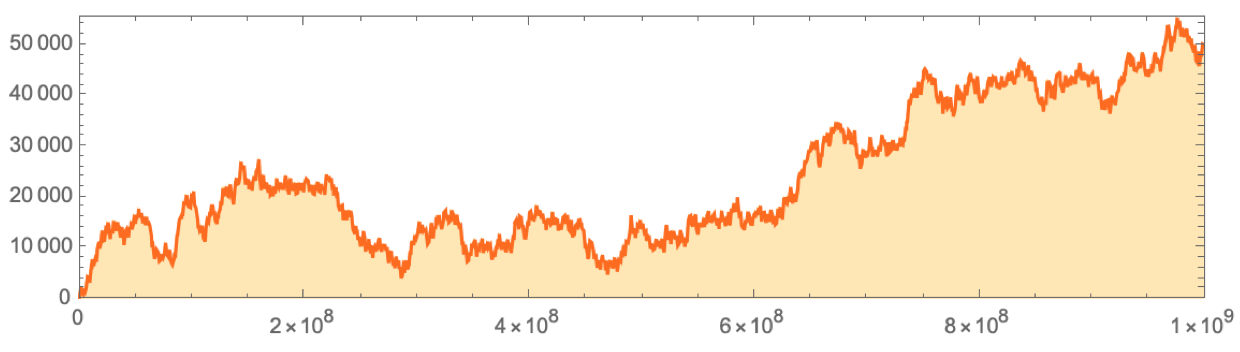✕ ```data=Flatten[IntegerDigits[#,2,8]&/@Normal[ResourceData["A Billion Bits of the Center Column of the Rule 30 Cellular Automaton"]]]; data=Accumulate[2 data-1]; sdata=Downsample[data,10^5]; ListLinePlot[Transpose[{Range 10^5,sdata}],Filling->Axis,Frame->True,PlotRangePadding->0,AspectRatio->1/4,MaxPlotPoints->1000,PlotStyle->Hue[0.07`,1,1],FillingStyle->Directive[Opacity[0.35`],Hue[0.12`,1,1]]]```

We can see that there are times when there’s an excess of 1s over 0s, and vice versa, though, yes, as we approach a billion steps 1 seems to be winning over 0, at least for now.

But let’s compute the ratio of the total number of 1s to the total number 0f 0s. Here’s what we get after 10,000 steps: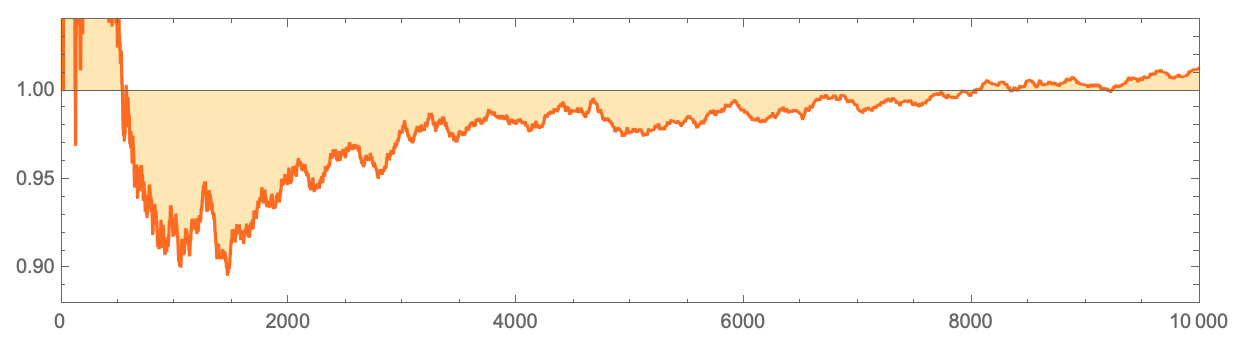✕ ```Quiet[ListLinePlot[ MapIndexed[#/(First[#2] - #) &, Accumulate[CellularAutomaton[30, {{1}, 0}, {10^4 - 1, {{0}}}]]], AspectRatio -> 1/4, Filling -> Axis, AxesOrigin -> {Automatic, 1}, Frame -> True, PlotRangePadding -> 0, PlotStyle -> Hue[0.07`, 1, 1], FillingStyle -> Directive[Opacity[0.35`], Hue[0.12`, 1, 1]], PlotRange -> {Automatic, {.88, 1.04}}]]```

Is this approaching the value 1? It’s hard to tell. Go on a little longer, and this is what we see: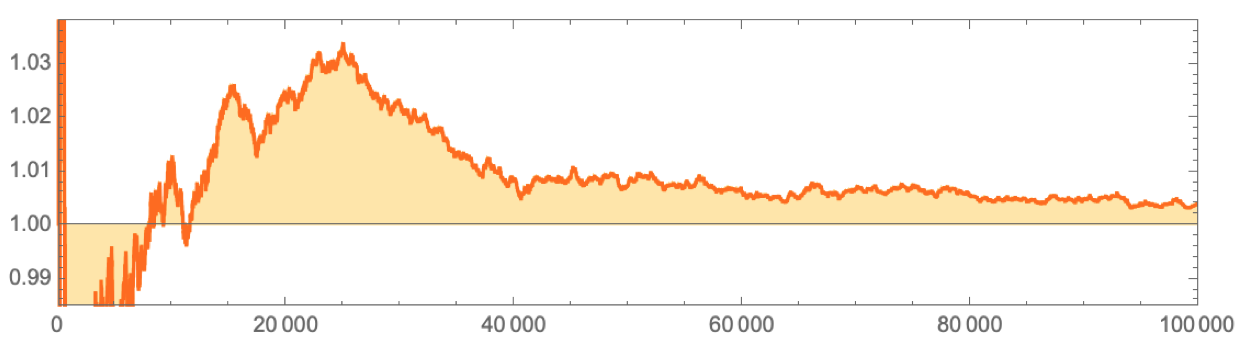✕ ```Quiet[ListLinePlot[ MapIndexed[#/(First[#2] - #) &, Accumulate[CellularAutomaton[30, {{1}, 0}, {10^5 - 1, {{0}}}]]], AspectRatio -> 1/4, Filling -> Axis, AxesOrigin -> {Automatic, 1}, Frame -> True, PlotRangePadding -> 0, PlotStyle -> Hue[0.07`, 1, 1], FillingStyle -> Directive[Opacity[0.35`], Hue[0.12`, 1, 1]], PlotRange -> {Automatic, {.985, 1.038}}]]```

The scale is getting smaller, but it’s still hard to tell what will happen. Plotting the difference from 1 on a log-log plot up to a billion steps suggests it’s fairly systematically getting smaller: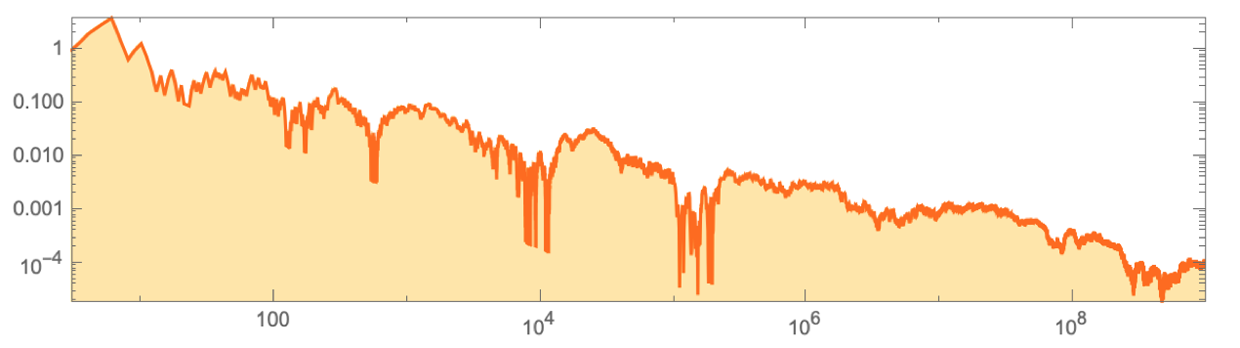✕ ```accdata=Accumulate[Flatten[IntegerDigits[#,2,8]&/@Normal[ResourceData["A Billion Bits of the Center Column of the Rule 30 Cellular Automaton"]]]]; diffratio=FunctionCompile[Function[Typed[arg,TypeSpecifier["PackedArray"]["MachineInteger",1]],MapIndexed[Abs[N[#]/(First[#2]-N[#])-1.]&,arg]]]; data=diffratio[accdata]; ListLogLogPlot[Join[Transpose[{Range[3,10^5],data[[3;;10^5]]}],Transpose[{Range[10^5+1000,10^9,1000],data[[10^5+1000;;10^9;;1000]]}]],Joined->True,AspectRatio->1/4,Frame->True,Filling->Axis,PlotRangePadding->0,PlotStyle->Hue[0.07`,1,1],FillingStyle->Directive[Opacity[0.35`],Hue[0.12`,1,1]]]```

But how do we know this trend will continue? Right now, we don’t. And, actually, things could get quite pathological. Maybe the fluctuations in 1s vs. 0s grow, so even though we’re averaging over longer and longer sequences, the overall ratio will never converge to a definite value.

Again, I doubt this is going to happen in the center column of rule 30. But without a proof, we don’t know for sure.

We’re asking here about the frequencies of black and white cells. But an obvious—and potentially illuminating—generalization is to ask instead about the frequencies for blocks of cells of length k. We can ask if all 2k such blocks have equal limiting frequency. Or we can ask the more basic question of whether all the blocks even ever occur—or, in other words, whether if one goes far enough, the center column of rule 30 will contain any given sequence of length k (say a bitwise representation of some work of literature).

Again, we can get empirical evidence. For example, at least up to k = 22, all 2k sequences do occur—and here’s how many steps it takes: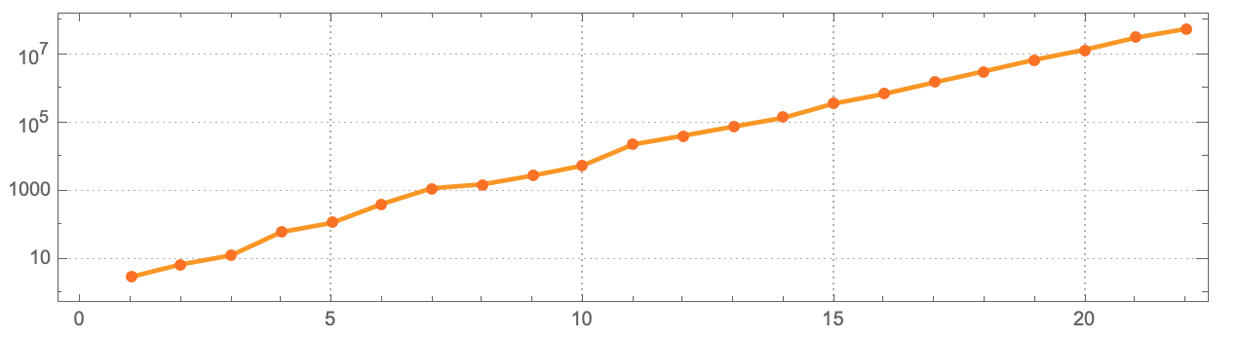✕ ```ListLogPlot[{3, 7, 13, 63, 116, 417, 1223, 1584, 2864, 5640, 23653, 42749, 78553, 143591, 377556, 720327, 1569318, 3367130, 7309616, 14383312, 32139368, 58671803}, Joined -> True, AspectRatio -> 1/4, Frame -> True, Mesh -> True, MeshStyle -> Directive[{Hue[0.07, 0.9500000000000001, 0.99], PointSize[.01]}], PlotTheme -> "Detailed", PlotStyle -> Directive[{Thickness[.004], Hue[0.1, 1, 0.99]}]]```

It’s worth noticing that one can succeed perfectly for blocks of one length, but then fail for larger blocks. For example, the Thue–Morse sequence mentioned above has exactly equal frequencies of 0 and 1, but pairs don’t occur with equal frequencies, and triples of identical elements simply never occur.

In traditional mathematics—and particularly dynamical systems theory—one approach to take is to consider not just evolution from a single-cell initial condition, but evolution from all possible initial conditions. And in this case it’s straightforward to show that, yes, if one evolves with equal probability from all possible initial conditions, then columns of cells generated by rule 30 will indeed contain every block with equal frequency. But if one asks the same thing for different distributions of initial conditions, one gets different results, and it’s not clear what the implication of this kind of analysis is for the specific case of a single-cell initial condition.

If different blocks occurred with different frequencies in the center column of rule 30, then that would immediately show that the center column is “not random”, or in other words that it has statistical regularities that could be used to at least statistically predict it. Of course, at some level the center column is completely “predictable”: you just have to run rule 30 to find it. But the question is whether, given just the values in the center column on their own, there’s a way to predict or compress them, say with much less computational effort than generating an arbitrary number of steps in the whole rule 30 pattern.

One could imagine running various data compression or statistical analysis algorithms, and asking whether they would succeed in finding regularities in the sequence. And particularly when one starts thinking about the overall computational capabilities of rule 30, it’s conceivable that one could prove something about how across a spectrum of possible analysis algorithms, there’s a limit to how much they could “reduce” the computation associated with the evolution of rule 30. But even given this, it’d likely still be a major challenge to say anything about the specific case of relative frequencies of black and white cells.

It’s perhaps worth mentioning one additional mathematical analog. Consider treating the values in a row of the rule 30 pattern as digits in a real number, say with the first digit of the fractional part being on the center column. Now, so far as we know, the evolution of rule 30 has no relation to any standard operations (like multiplication or taking powers) that one does on real numbers. But we can still ask about the sequence of numbers formed by looking at the right-hand side of the rule 30 pattern. Here’s a plot for the first 200 steps: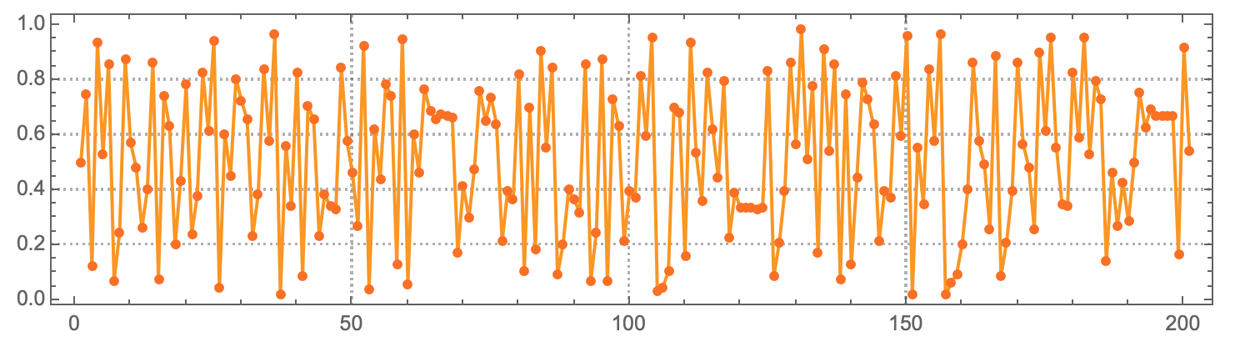✕ ```ListLinePlot[ FromDigits[{#, 0}, 2] & /@ CellularAutomaton[30, {{1}, 0}, {200, {0, 200}}], Mesh -> All, AspectRatio -> 1/4, Frame -> True, MeshStyle -> Directive[{Hue[0.07, 0.9500000000000001, 0.99], PointSize[.0085]}], PlotTheme -> "Detailed", PlotStyle -> Directive[{ Hue[0.1, 1, 0.99]}], ImageSize -> 575]```

And here’s a histogram of the values reached at successively more steps: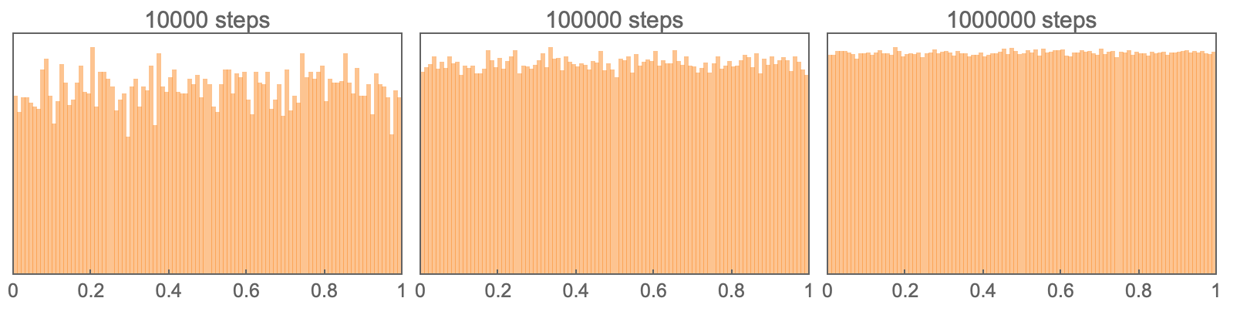✕ ```Grid[{Table[ Histogram[ FromDigits[{#, 0}, 2] & /@ CellularAutomaton[30, {{1}, 0}, {10^n, {0, 20}}], {.01}, Frame -> True, FrameTicks -> {{None, None}, {{{0, "0"}, .2, .4, .6, .8, {1, "1"}}, None}}, PlotLabel -> (StringTemplate["`` steps"][10^n]), ChartStyle -> Directive[Opacity[.5], Hue[0.09, 1, 1]], ImageSize -> 208, PlotRangePadding -> {{0, 0}, {0, Scaled[.06]}}], {n, 4, 6}]}, Spacings -> .2]```

And, yes, it’s consistent with the limiting histogram being flat, or in other words, with these numbers being uniformly distributed in the interval 0 to 1.

Well, it turns out that in the early 1900s there were a bunch of mathematical results established about this kind of equidistribution. In particular, it’s known that FractionalPart[h n] for successive n is always equidistributed if h isn’t a rational number. It’s also known that FractionalPart[hn] is equidistributed for almost all h (Pisot numbers like the golden ratio are exceptions). But specific cases—like FractionalPart[(3/2)n]—have eluded analysis for at least half a century. (By the way, it’s known that the digits of π in base 16 and thus base 2 can be generated by a recurrence of the form xn = FractionalPart[16 xn-1 + r[n]] where r[n] is a fixed rational function of n.)

### Problem 3: Does computing the nth cell of the center column require at least O(n) computational effort?

Consider the pattern made by rule 150: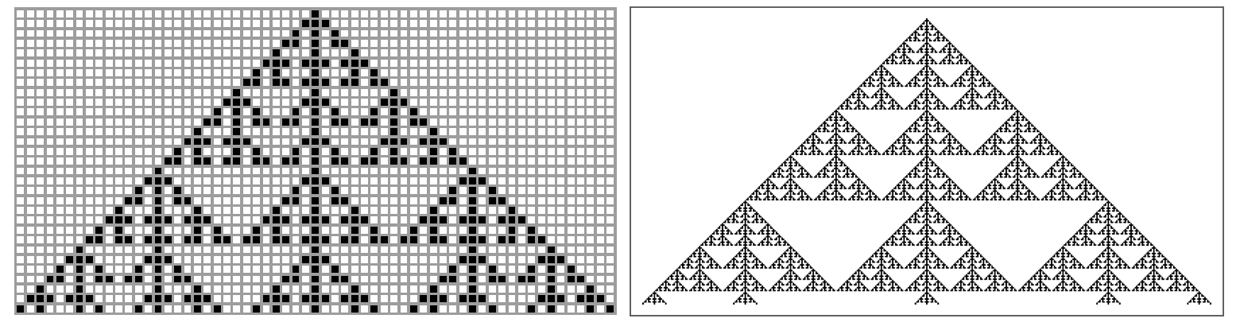✕ ```Row[{ArrayPlot[CellularAutomaton[150, {{1}, 0}, 30], Mesh -> All, ImageSize -> 315], ArrayPlot[CellularAutomaton[150, {{1}, 0}, 200], ImageSize -> 300]}]```

It’s a very regular, nested pattern. Its center column happens to be trivial (all cells are black). But if we look one column to the left or right, we find: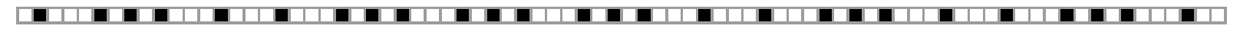✕ ```ArrayPlot[{Table[Mod[IntegerExponent[t, 2], 2], {t, 80}]}, Mesh -> All, ImageSize -> Full]```

How do we work out the value of the nth cell? Well, in this particular case, it turns out there’s essentially just a simple formula: the value is given by Mod[IntegerExponent[n, 2], 2]. In other words, just look at the number n in base 2, and ask whether the number of zeros it has at the end is even or odd.

How much computational effort does it take to “evaluate this formula”? Well, even if we have to check every bit in n, there are only about Log[2, n] of those. So we can expect that the computational effort is O(log n).

But what about the rule 30 case? We know we can work out the value of the nth cell in the center column just by explicitly applying the rule 30 update rule n2 times. But the question is whether there’s a way to reduce the computational work that’s needed. In the past, there’s tended to be an implicit assumption throughout the mathematical sciences that if one has the right model for something, then by just being clever enough one will always find a way to make predictions—or in other words, to work out what a system will do, using a lot less computational effort than the actual evolution of the system requires.

And, yes, there are plenty of examples of “exact solutions” (think 2-body problem, 2D Ising model, etc.) where we essentially just get a formula for what a system will do. But there are also other cases (think 3-body problem, 3D Ising model, etc.) where this has never successfully been done.

And as I first discussed in the early 1980s, I suspect that there are actually many systems (including these) that are computationally irreducible, in the sense that there’s no way to significantly reduce the amount of computational work needed to determine their behavior.

So in effect Problem 3 is asking about the computational irreducibility of rule 30—or at least a specific aspect of it. (The choice of O(n) computational effort is somewhat arbitrary; another version of this problem could ask for O(nα) for any α<2, or, for that matter, O(log β(n))—or some criterion based on both time and memory resources.)

If the answer to Problem 3 is negative, then the obvious way to show this would just be to give an explicit program that successfully computes the nth value in the center column with less than O(n) computational effort, as we did for rule 150 above.

We can ask what O(n) computational effort means. What kind of system are we supposed to use to do the computation? And how do we measure “computational effort”? The phenomenon of computational universality implies that—within some basic constraints—it ultimately doesn’t matter.

For definiteness we could say that we always want to do the computation on a Turing machine. And for example we can say that we’ll feed the digits of the number n in as the initial state of the Turing machine tape, then expect the Turing machine to grind for much less than n steps before generating the answer (and, if it’s really to be “formula like”, more like O(log n) steps).

We don’t need to base things on a Turing machine, of course. We could use any kind of system capable of universal computation, including a cellular automaton, and, for that matter, the whole Wolfram Language. It gets a little harder to measure “computational effort” in these systems. Presumably in a cellular automaton we’d want to count the total number of cell updates done. And in the Wolfram Language we might end up just actually measuring CPU time for executing whatever program we’ve set up.

I strongly suspect that rule 30 is computationally irreducible, and that Problem 3 has an affirmative answer. But if isn’t, my guess is that eventually there’ll turn out to be a program that rather obviously computes the nth value in less than O(n) computational effort, and there won’t be a lot of argument about the details of whether the computational resources are counted correctly.

But proving that no such program exists is a much more difficult proposition. And even though I suspect computational irreducibility is quite ubiquitous, it’s always very hard to prove explicit lower bounds on the difficulty of doing particular computations. And in fact almost all explicit lower bounds currently known are quite weak, and essentially boil down just to arguments about information content—like that you need O(log n) steps to even read all the digits in the value of n.

Undoubtedly the most famous lower-bound problem is the P vs. NP question. I don’t think there’s a direct relation to our rule 30 problem (which is more like a P vs. LOGTIME question), but it’s perhaps worth understanding how things are connected. The basic point is that the forward evolution of a cellular automaton, say for n steps from an initial condition with n cells specified, is at most an O(n2) computation, and is therefore in P (“polynomial time”). But the question of whether there exists an initial condition that evolves to produce some particular final result is in NP. If you happen (“non-deterministically”) to pick the correct initial condition, then it’s polynomial time to check that it’s correct. But there are potentially 2n possible initial conditions to check.

Of course there are plenty of cellular automata where you don’t have to check all these 2n initial conditions, and a polynomial-time computation clearly suffices. But it’s possible to construct a cellular automaton where finding the initial condition is an NP-complete problem, or in other words, where it’s possible to encode any problem in NP in this particular cellular automaton inversion problem. Is the rule 30 inversion problem NP-complete? We don’t know, though it seems conceivable that it could be proved to be (and if one did prove it then rule 30 could finally be a provably NP-complete cryptosystem).

But there doesn’t seem to be a direct connection between the inversion problem for rule 30, and the problem of predicting the center column. Still, there’s at least a more direct connection to another global question: whether rule 30 is computation universal, or, in other words, whether there exist initial conditions for rule 30 that allow it to be “programmed” to perform any computation that, for example, any Turing machine can perform.

We know that among the 256 simplest cellular automata, rule 110 is universal (as are three other rules that are simple transformations of it). But looking at a typical example of rule 110 evolution, it’s already clear that there are definite, modular structures one can identify. And indeed the proof proceeds by showing how one can “engineer” a known universal system out of rule 110 by appropriately assembling these structures.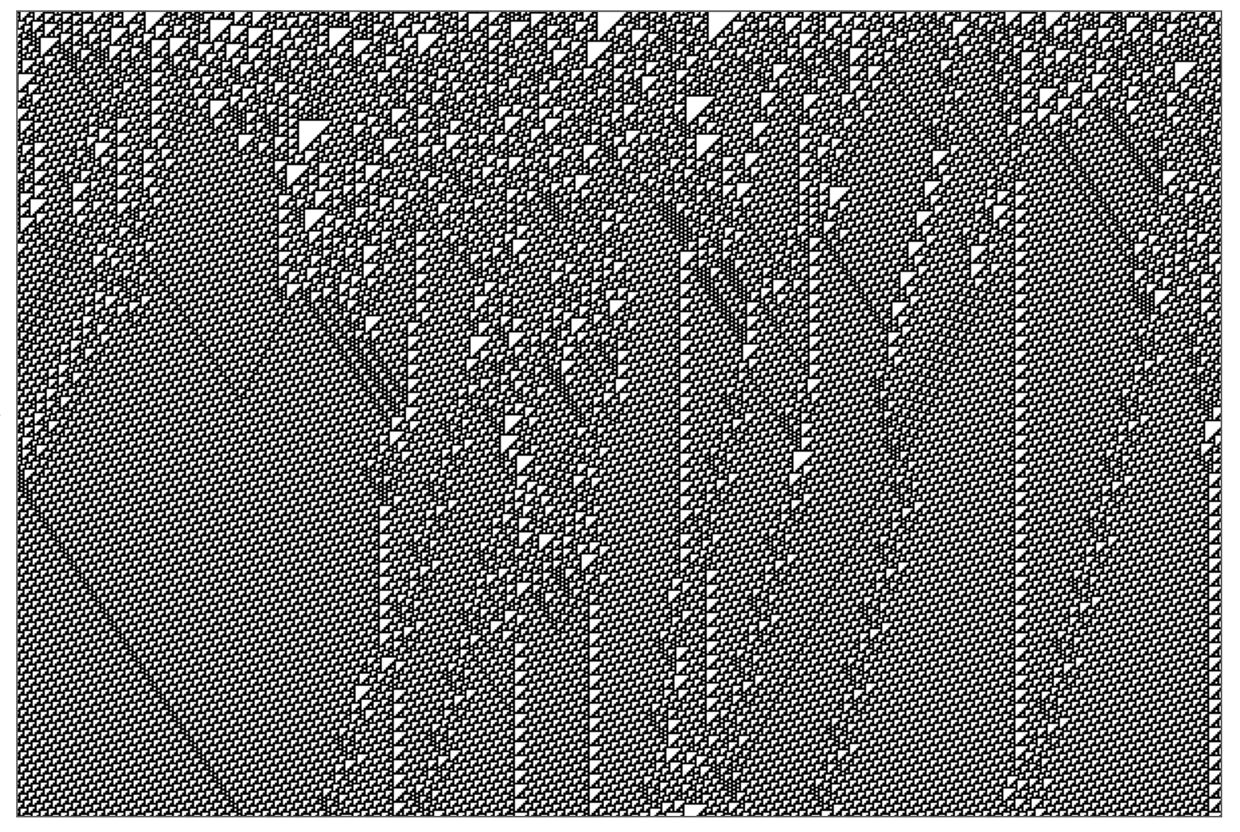✕ ```SeedRandom; ArrayPlot[ CellularAutomaton[110, RandomInteger[1, 600], 400], PixelConstrained -> 1]```

Rule 30, however, shows no such obvious modularity—so it doesn’t seem plausible that one can establish universality in the “engineering” way it’s been established for all other known-to-be-universal systems. Still, my Principle of Computational Equivalence strongly suggests that rule 30 is indeed universal; we just don’t yet have an obvious direction to take in trying to prove it.

If one can show that a system is universal, however, then this does have implications that are closer to our rule 30 problem. In particular, if a system is universal, then there’ll be questions (like the halting problem) about its infinite-time behavior that will be undecidable, and which no guaranteed-finite-time computation can answer. But as such, universality is a statement about the existence of initial conditions that reproduce a given computation. It doesn’t say anything about the specifics of a particular initial condition—or about how long it will take to compute a particular result.

OK, but what about a different direction: what about getting empirical evidence about our Problem 3? Is there a way to use statistics, or cryptanalysis, or mathematics, or machine learning to even slightly reduce the computational effort needed to compute the nth value in the center column?

Well, we know that the whole 2D pattern of rule 30 is far from random. In fact, of all 2m2 patches, only m × m can possibly occur—and in practice the number weighted by probability is much smaller. And I don’t doubt that facts like this can be used to reduce the effort to compute the center column to less than O(n2) effort (and that would be a nice partial result). But can it be less than O(n) effort? That’s a much more difficult question.

Clearly if Problem 1 was answered in the negative then it could be. But in a sense asking for less than O(n) computation of the center column is precisely like asking whether there are “predictable regularities” in it. Of course, even if one could find small-scale statistical regularities in the sequence (as answering Problem 2 in the negative would imply), these wouldn’t on their own give one a way to do more than perhaps slightly improve a constant multiplier in the speed of computing the sequence.

Could there be some systematically reduced way to compute the sequence using a neural net—which is essentially a collection of nested real-number functions? I’ve tried to find such a neural net using our current deep-learning technology—and haven’t been able to get anywhere at all.

What about statistical methods? If we could find statistical non-randomness in the sequence, then that would imply an ability to compress the sequence, and thus some redundancy or predictability in the sequence. But I’ve tried all sorts of statistical randomness tests on the center column of rule 30—and never found any significant deviation from randomness. (And for many years—until we found a slightly more efficient rule—we used sequences from finite-size rule 30 systems as our source of random numbers in the Wolfram Language, and no legitimate “it’s not random!” bugs ever showed up.)

Statistical tests of randomness typically work by saying, “Take the supposedly random sequence and process it in some way, then see if the result is obviously non-random”. But what kind of processing should be done? One might see if blocks occur with equal frequency, or if correlations exist, or if some compression algorithm succeeds in doing compression. But typically batteries of tests end up seeming a bit haphazard and arbitrary. In principle one can imagine enumerating all possible tests—by enumerating all possible programs that can be applied to the sequence. But I’ve tried doing this, for example for classes of cellular automaton rules—and have never managed to detect any non-randomness in the rule 30 sequence.

So how about using ideas from mathematics to predict the rule 30 sequence? Well, as such, rule 30 doesn’t seem connected to any well-developed area of math. But of course it’s conceivable that some mapping could be found between rule 30 and ideas, say, in an area like number theory—and that these could either help in finding a shortcut for computing rule 30, or could show that computing it is equivalent to some problem like integer factoring that’s thought to be fundamentally difficult.

I know a few examples of interesting interplays between traditional mathematical structures and cellular automata. For example, consider the digits of successive powers of 3 in base 2 and in base 6: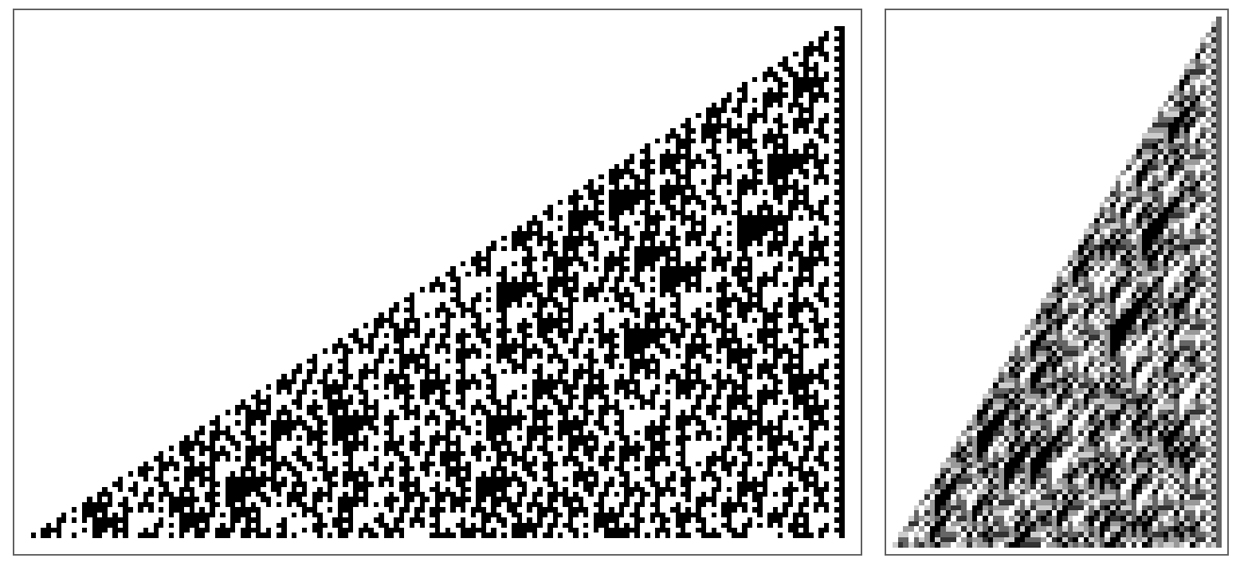✕ ```Row[Riffle[ ArrayPlot[#, ImageSize -> {Automatic, 275}] & /@ {Table[ IntegerDigits[3^t, 2, 159], {t, 100}], Table[IntegerDigits[3^t, 6, 62], {t, 100}]}, Spacer]]```

It turns out that in the base 6 case, the rule for generating the pattern is exactly a cellular automaton. (For base 2, there are additional long-range carries.) But although both these patterns look complex, it turns out that their mathematical structure lets us speed up making certain predictions about them.

Consider the sth digit from the right-hand edge of line n in each pattern. It’s just the sth digit in 3n, which is given by the “formula” (where b is the base, here 2 or 6) Mod[Quotient[3n, bs], b]. But how easy is it to evaluate this formula? One might think that to compute 3n one would have to do n multiplications. But this isn’t the case: instead, one can for example build up 3n using repeated squaring, with about log(n) multiplications. That this is possible is a consequence of the associativity of multiplication. There’s nothing obviously like that for rule 30—but it’s always conceivable that some mapping to a mathematical structure like this could be found.

Talking of mathematical structure, it’s worth mentioning that there are more formula-like ways to state the basic rule for rule 30. For example, taking the values of three adjacent cells to be p, q, r the basic rule is just p (q r) or Xor[p, Or[q, r]]. With numerical cell values 0 and 1, the basic rule is just Mod[p + q + r + q r, 2]. Do these forms help? I don’t know. But, for example, it’s remarkable that in a sense all the complexity of rule 30 comes from the presence of that one little nonlinear q r term—for without that term, one would have rule 150, about which one can develop a complete algebraic theory using quite traditional mathematics.

To work out n steps in the evolution of rule 30, one’s effectively got to repeatedly compose the basic rule. And so far as one can tell, the symbolic expressions that arise just get more and more complicated—and don’t show any sign of simplifying in such a way as to save computational work.

In Problem 3, we’re talking about the computational effort to compute the nth value in the center column of rule 30—and asking if it can be less than O(n). But imagine that we have a definite algorithm for doing the computation. For any given n, we can see what computational resources it uses. Say the result is r[n]. Then what we’re asking is whether r[n] is less than “big O” of n, or whether MaxLimit[r[n]/n, n ]<.

But imagine that we have a particular Turing machine (or some other computational system) that’s implementing our algorithm. It could be that r[n] will at least asymptotically just be a smooth or otherwise regular function of n for which it’s easy to see what the limit is. But if one just starts enumerating Turing machines, one encounters examples where r[n] appears to have peaks of random heights in random places. It might even be that somewhere there’d be a value of n for which the Turing machine doesn’t halt (or whatever) at all, so that r[n] is infinite. And in general, as we’ll discuss in more detail later, it could even be undecidable just how r[n] grows relative to O(n).

## Formal Statements of the Problems

So far, I’ve mostly described the Prize Problems in words. But we can also describe them in computational language (or effectively also in math).

In the Wolfram Language, the first t values in the center column of rule 30 are given by: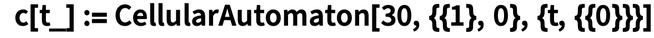✕ `c[t_] := CellularAutomaton[30, {{1}, 0}, {t, {{0}}}]`

And with this definition, the three problems can be stated as predicates about c[t].

### Problem 1: Does the center column always remain non-periodic?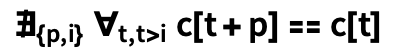✕ ```\!\( \*SubscriptBox[\(\[NotExists]\), \({p, i}\)]\( \*SubscriptBox[\(\[ForAll]\), \(t, t > i\)]c[t + p] == c[t]\)\)```

or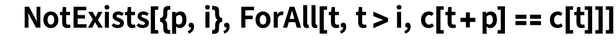✕ `NotExists[{p, i}, ForAll[t, t > i, c[t + p] == c[t]]]`

or “there does not exist a period p and an initial length i such that for all t with t>i, c[t + p] equals c[t]”.

### Problem 2: Does each color of cell occur on average equally often in the center column?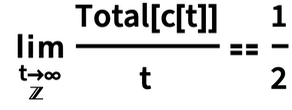✕ ```\!\(\*UnderscriptBox[\(\[Limit]\), \(t\* UnderscriptBox["\[Rule]", TemplateBox[{}, "Integers"]]\[Infinity]\)]\) Total[c[t]]/t == 1/2```

or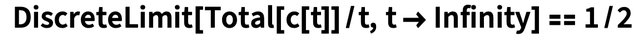✕ `DiscreteLimit[Total[c[t]]/t, t -> Infinity] == 1/2`

or “the discrete limit of the total of the values in c[t]/t as t is 1/2”.

### Problem 3: Does computing the nth cell of the center column require at least O(n) computational effort?

Define machine[m] to be a machine parametrized by m (for example TuringMachine[...]), and let machine[m][n] give {v, t}, where v is the output value, and t is the amount of computational effort taken (e.g. number of steps). Then the problem can be formulated as: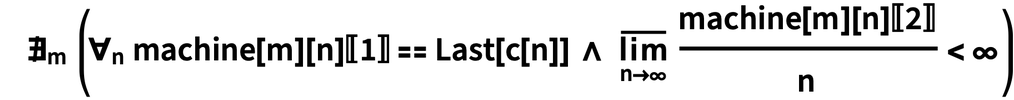✕ ```\!\( \*SubscriptBox[\(\[NotExists]\), \(m\)]\(( \*SubscriptBox[\(\[ForAll]\), \(n\)]\(\(machine[m]\)[n]\)[] == Last[c[n]]\ \[And] \ \*UnderscriptBox[\(\[MaxLimit]\), \(n -> \[Infinity]\)] \*FractionBox[\(\(\(machine[m]\)[n]\)[[ 2]]\), \(n\)] < \[Infinity])\)\)```

or “there does not exist a machine m which for all n gives c[n], and for which the lim sup of the amount of computational effort spent, divided by n, is finite”. (Yes, one should also require that m be finite, so the machine’s rule can’t just store the answer.)

## The Formal Character of Solutions

Before we discuss the individual problems, an obvious question to ask is what the interdependence of the problems might be. If the answer to Problem 3 is negative (which I very strongly doubt), then it holds the possibility for simple algorithms or formulas from which the answers to Problems 1 and 2 might become straightforward. If the answer to Problem 3 is affirmative (as I strongly suspect), then it implies that the answer to Problem 1 must also be affirmative. The contrapositive is also true: if the answer to Problem 1 is negative, then it implies that the answer to Problem 3 must also be negative.

If the answer to Problem 1 is negative, so that there is some periodic sequence that appears in the center column, then if one explicitly knows that sequence, one can immediately answer Problem 2. One might think that answering Problem 2 in the negative would imply something about Problem 3. And, yes, unequal probabilities for black and white implies compression by a constant factor in a Shannon-information way. But to compute value with less than O(n) resources—and therefore to answer Problem 3 in the negative—requires that one be able to identify in a sense infinitely more compression.

So what does it take to establish the answers to the problems?

If Problem 1 is answered in the negative, then one can imagine explicitly exhibiting the pattern generated by rule 30 at some known step—and being able to see the periodic sequence in the center. Of course, Problem 1 could still be answered in the negative, but less constructively. One might be able to show that eventually the sequence has to be periodic, but not know even any bound on where this might happen. If Problem 3 is answered in the negative, a way to do this is to explicitly give an algorithm (or, say, a Turing machine) that does the computation with less than O(n) computational resources.

But let’s say one has such an algorithm. One still has to prove that for all n, the algorithm will correctly reproduce the nth value. This might be easy. Perhaps there would just be a proof by induction or some such. But it might be arbitrarily hard. For example, it could be that for most n, the running time of the algorithm is clearly less than n. But it might not be obvious that the running time will always even be finite. Indeed, the “halting problem” for the algorithm might simply be undecidable. But just showing that a particular algorithm doesn’t halt for a given n doesn’t really tell one anything about the answer to the problem. For that one would have to show that there’s no algorithm that exists that will successfully halt in less than O(n) time.

The mention of undecidability brings up an issue, however: just what axiom system is one supposed to use to answer the problems? For the purposes of the Prize, I’ll just say “the traditional axioms of standard mathematics”, which one can assume are Peano arithmetic and/or the axioms of set theory (with or without the continuum hypothesis).

Could it be that the answers to the problems depend on the choice of axioms—or even that they’re independent of the traditional axioms (in the sense of Gödel’s incompleteness theorem)? Historical experience in mathematics makes this seem extremely unlikely, because, to date, essentially all “natural” problems in mathematics seem to have turned out to be decidable in the (sometimes rather implicit) axiom system that’s used in doing the mathematics.

In the computational universe, though—freed from the bounds of historical math tradition—it’s vastly more common to run into undecidability. And, actually, my guess is that a fair fraction of long-unsolved problems even in traditional mathematics will also turn out to be undecidable. So that definitely raises the possibility that the problems here could be independent of at least some standard axiom systems.

OK, but assume there’s no undecidability around, and one’s not dealing with the few cases in which one can just answer a problem by saying “look at this explicitly constructed thing”. Well, then to answer the problem, we’re going to have to give a proof.

In essence what drives the need for proof is the presence of something infinite. We want to know something for any n, even infinitely large, etc. And the only way to handle this is then to represent things symbolically (“the symbol Infinity means infinity”, etc.), and apply formal rules to everything, defined by the axioms in the underlying axiom system one’s assuming.

In the best case, one might be able to just explicitly exhibit that series of rule applications—in such a way that a computer can immediately verify that they’re correct. Perhaps the series of rule applications could be found by automated theorem proving (as in FindEquationalProof). More likely, it might be constructed using a proof assistant system.

It would certainly be exciting to have a fully formalized proof of the answer to any of the problems. But my guess is that it’ll be vastly easier to construct a standard proof of the kind human mathematicians traditionally do. What is such a proof? Well, it’s basically an argument that will convince other humans that a result is correct.

There isn’t really a precise definition of that. In our step-by-step solutions in Wolfram|Alpha, we’re effectively proving results (say in calculus) in such a way that students can follow them. In an academic math journal, one’s giving proofs that successfully get past the peer review process for the journal.

My own guess would be that if one were to try to formalize essentially any nontrivial proof in the math literature, one would find little corners that require new results, though usually ones that wouldn’t be too hard to get.

How can we handle this in practice for our prizes? In essence, we have to define a computational contract for what constitutes success, and when prize money should be paid out. For a constructive proof, we can get Wolfram Language code that can explicitly be run on any sufficiently large computer to establish the result. For formalized proofs, we can get Wolfram Language code that can run through the proof, validating each step.

But what about for a “human proof”? Ultimately we have no choice but to rely on some kind of human review process. We can ask multiple people to verify the proof. We could have some blockchain-inspired scheme where people “stake” the correctness of the proof, then if one eventually gets consensus (whatever this means) one pays out to people some of the prize money, in proportion to their stake. But whatever is done, it’s going to be an imperfect, “societal” result—like almost all of the pure mathematics that’s so far been done in the world.

## What Will It Take?

OK, so for people interested in working on the Problems, what skills are relevant? I don’t really know. It could be discrete and combinatorial mathematics. It could be number theory, if there’s a correspondence with number-based systems found. It could be some branch of algebraic mathematics, if there’s a correspondence with algebraic systems found. It could be dynamical systems theory. It could be something closer to mathematical logic or theoretical computer science, like the theory of term rewriting systems.

Of course, it could be that no existing towers of knowledge—say in branches of mathematics—will be relevant to the problems, and that to solve them will require building “from the ground up”. And indeed that’s effectively what ended up happening in the solution for my 2,3 Turing Machine Prize in 2007.

I’m a great believer in the power of computer experiments—and of course it’s on the basis of computer experiments that I’ve formulated the Rule 30 Prize Problems. But there are definitely more computer experiments that could be done. So far we know a billion elements in the center column sequence. And so far the sequence doesn’t seem to show any deviation from randomness (at least based on tests I’ve tried). But maybe at a trillion elements (which should be well within range of current computer systems) or a quadrillion elements, or more, it eventually will—and it’s definitely worth doing the computations to check.

The direct way to compute n elements in the center column is to run rule 30 for n steps, using at an intermediate stage up to n cells of memory. The actual computation is quite well optimized in the Wolfram Language. Running on my desktop computer, it takes less than 0.4 seconds to compute 100,000 elements: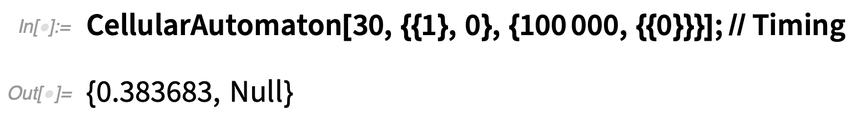✕ `CellularAutomaton[30, {{1}, 0}, {100000, {{0}}}]; // Timing`

Internally, this is using the fact that rule 30 can be expressed as Xor[p, Or[q, r]], and implemented using bitwise operations on whole words of data at a time. Using explicit bitwise operations on long integers takes about twice as long as the built-in CellularAutomaton function: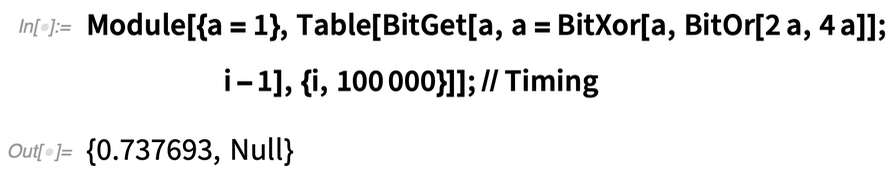✕ ```Module[{a = 1}, Table[BitGet[a, a = BitXor[a, BitOr[2 a, 4 a]]; i - 1], {i, 100000}]]; // Timing```

But these results are from single CPU processors. It’s perfectly possible to imagine parallelizing across many CPUs, or using GPUs. One might imagine that one could speed up the computation by effectively caching the results of many steps in rule 30 evolution, but the fact that across the rows of the rule 30 pattern all blocks appear to occur with at least roughly equal frequency makes it seem as though this would not lead to significant speedup.

Solving some types of math-like problems seem pretty certain to require deep knowledge of high-level existing mathematics. For example, it seems quite unlikely that there can be an “elementary” proof of Fermat’s last theorem, or even of the four-color theorem. But for the Rule 30 Prize Problems it’s not clear to me. Each of them might need sophisticated existing mathematics, or they might not. They might be accessible only to people professionally trained in mathematics, or they might be solvable by clever “programming-style” or “puzzle-style” work, without sophisticated mathematics.

## Generalizations and Relations

Sometimes the best way to solve a specific problem is first to solve a related problem—often a more general one—and then come back to the specific problem. And there are certainly many problems related to the Rule 30 Prize Problems that one can consider.

For example, instead of looking at the vertical column of cells at the center of the rule 30 pattern, one could look at a column of cells in a different direction. At 45°, it’s easy to see that any sequence must be periodic. On the left the periods increase very slowly; on the right they increase rapidly. But what about other angles?

Or what about looking at rows of cells in the pattern? Do all possible blocks occur? How many steps is it before any given block appears? The empirical evidence doesn’t see any deviation from blocks occurring at random, but obviously, for example, successive rows are highly correlated.

What about different initial conditions? There are many dynamical systems–style results about the behavior of rule 30 starting with equal probability from all possible infinite initial conditions. In this case, for example, it’s easy to show that all possible blocks occur with equal frequency, both at a given row, and in a given vertical column. Things get more complicated if one asks for initial conditions that correspond, for example, to all possible sequences generated by a given finite state machine, and one could imagine that from a sequence of results about different sets of possible initial conditions, one would eventually be able to say something about the case of the single black cell initial condition.

Another straightforward generalization is just to look not at a single black cell initial condition, but at other “special” initial conditions. An infinite periodic initial condition will always give periodic behavior (that’s the same as one gets in a finite-size region with periodic boundary conditions). But one can, for example, study what happens if one puts a “single defect” in the periodic pattern: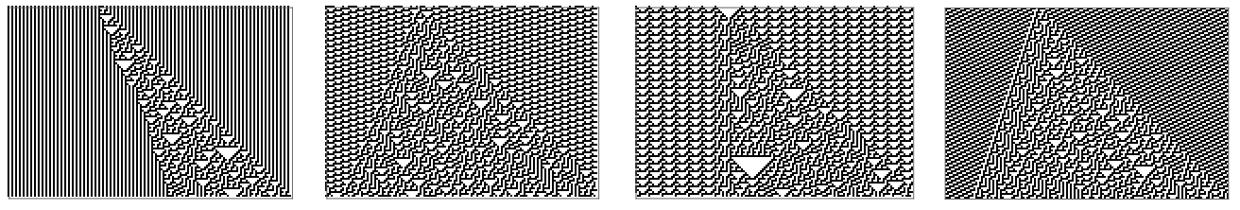✕ ```GraphicsRow[(ArrayPlot[ CellularAutomaton[30, MapAt[1 - #1 &, Flatten[Table[#1, Round[150/Length[#1]]]], 50], 100]] &) /@ {{1, 0}, {1, 1, 1, 1, 1, 0, 0, 0, 0, 1, 0, 0}, {1, 0, 0, 0, 0, 0, 0}, {1, 1, 1, 0, 0}}]```

One can also ask what happens when one has not just a single black cell, but some longer sequence in the initial conditions. How does the center column change with different initial sequences? Are there finite initial sequences that lead to “simpler” center columns?

Or are there infinite initial conditions generated by other computational systems (say substitution systems) that aren’t periodic, but still give somehow simple rule 30 patterns?

Then one can imagine going “beyond” rule 30. What happens if one adds longer-range “exceptions” to the rules? When do extensions to rule 30 show behavior that can be analyzed in one way or another? And can one then see the effect of removing the “exceptions” in the rule?

Of course, one can consider rules quite different from rule 30 as well—and perhaps hope to develop intuition or methods relevant to rule 30 by looking at other rules. Even among the 256 two-color nearest-neighbor rules, there are others that show complex behavior starting from a simple initial condition: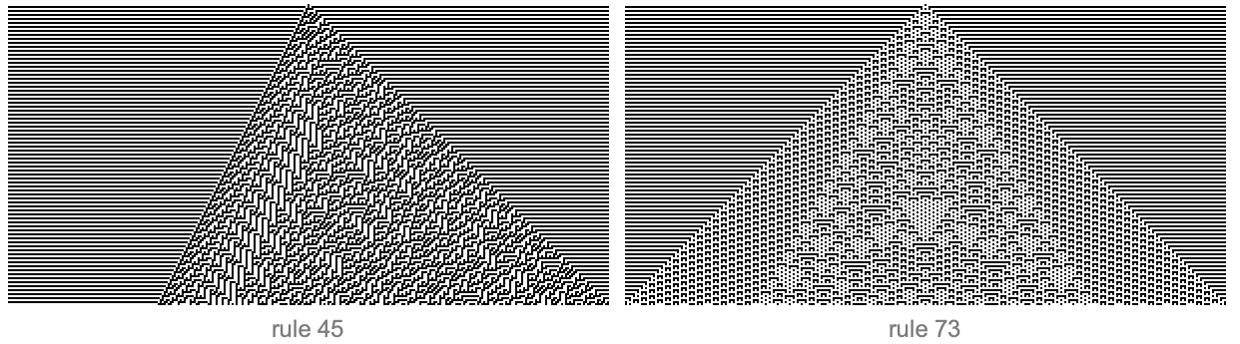✕ ```Row[Riffle[ Labeled[ArrayPlot[CellularAutomaton[#, {{1}, 0}, {150, All}], PixelConstrained -> 1, Frame -> False], Style[Text[StringTemplate["rule ``"][#]], 12], LabelStyle -> Opacity[.5]] & /@ {45, 73}, Spacer]]```

And if one looks at larger numbers of colors and larger neighbors one can find an infinite number of examples. There’s all sorts of behavior that one sees. And, for example, given any particular sequence, one can search for rules that will generate it as their center column. One can also try to classify the center-column sequences that one sees, perhaps identifying a general class “like rule 30” about which global statements can be made.

But let’s discuss the specific Rule 30 Prize Problems. To investigate the possibility of periodicity in rule 30 (as in Problem 1), one could study lots of different rules, looking for examples with very long periods, or very long transients—and try to use these to develop an intuition for how and when these can occur.

To investigate the equal-frequency phenomenon of Problem 2, one can look at different statistical features, and see both in rule 30 and across different rules when it’s possible to detect regularity.

For Problem 3, one can start looking at different levels of computational effort. Can one find the nth value with computational effort O(nγ) for any γ<2 (I don't know any method to achieve this)? Can one show that one can’t find the nth value with less than O(log(n)) computational effort? What about with less than O(log(n)) available memory? What about for different rules? Periodic and nested patterns are easy to compute quickly. But what other examples can one find?

As I’ve mentioned, a big achievement would be to show computation universality for rule 30. But even if one can’t do it for rule 30, finding additional examples (beyond, for example, rule 110) will help build intuition about what might be going on in rule 30.

Then there’s NP-completeness. Is there a way of setting up some question about the behavior of rule 30 for some family of initial conditions where it’s possible to prove that the question is NP-complete? If this worked, it would be an exciting result for cryptography. And perhaps, again, one can build up intuition by looking at other rules, even ones that are more “purposefully constructed” than rule 30.

## How Hard Are the Problems?

When I set up my 2,3 Turing Machine Prize in 2007 I didn’t know if it’d be solved in a month, a year, a decade, a century, or more. As it turned out, it was actually solved in about four months. So what will happen with the Rule 30 Prize Problems? I don’t know. After nearly 40 years, I’d be surprised if any of them could now be solved in a month (but it’d be really exciting if that happened!). And of course some superficially similar problems (like features of the digits of π) have been out there for well over a century.

It’s not clear whether there’s any sophisticated math (or computer science) that exists today that will be helpful in solving the problems. But I’m confident that whatever is built to solve them will provide structure that will be important for solving other problems about the computational universe. And the longer it takes (think Fermat’s last theorem), the larger the amount of useful structure is likely to be built on the way to a solution.

I don’t know if solutions to the problems will be “obviously correct” (it’ll help if they’re constructive, or presented in computable form), or whether there’ll be a long period of verification to go through. I don’t know if proofs will be comparatively short, or outrageously long. I don’t know if the solutions will depend on details of axiom systems (“assuming the continuum hypothesis”, etc.), or if they’ll be robust for any reasonable choices of axioms. I don’t know if the three problems are somehow “comparably difficult”—or if one or two might be solved, with the others holding out for a very long time.

But what I am sure about is that solving any of the problems will be a significant achievement. I’ve picked the problems to be specific, definite and concrete. But the issues of randomness and computational irreducibility that they address are deep and general. And to know the solutions to these problems will provide important evidence and raw material for thinking about these issues wherever they occur.

Of course, having lived now with rule 30 and its implications for nearly 40 years, I will personally be thrilled to know for certain even a little more about its remarkable behavior.

To comment, please visit the copy of this post at Stephen Wolfram’s Writings »